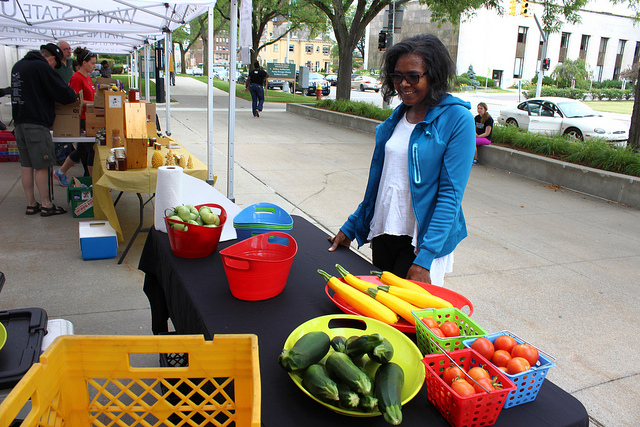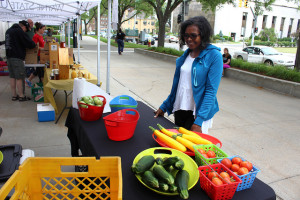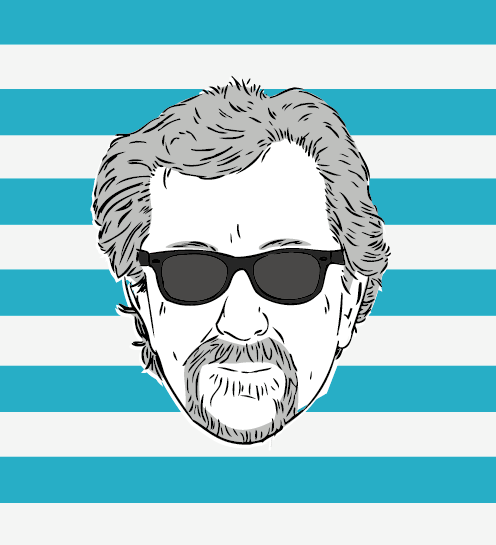Sound Bites: You Aren’t Dangerous Until You Can Speak Powerfully | Perdue Farms Buys Niman Ranch// <![CDATA[ var GM_START_TIME=(new Date).getTime();var GM_SUPPORTED_IE_VERSION="9.0";var GM_SUPPORTED_GECKO_VERSION="2.0.0";var GM_MOOSE_URL="?ui\x3dhtml\x26zy\x3db";var GM_NO_COOKIE_URL="?view\x3dnocookies";var GM_NO_ACTIVEX_URL="?view\x3dnoactivex";var GM_APP_NAME="Gmail";var GM_ACTION_TOKEN="AF6bupMotDRRoaoEnz_Oswc0FeYRtPnA-g"; // ]]&gt;// <![CDATA[ (function(){try{var h=this,aa=Date.now||function(){return+new Date};var k=String.prototype.trim?function(a){return a.trim()}:function(a){return a.replace(/^[\s\xa0]+|[\s\xa0]+\$/g,"")},l=function(a,b){return-1!=a.indexOf(b)},m=function(a,b){return a<b?-1:a>b?1:0};var n;a:{var p=h.navigator;if(p){var q=p.userAgent;if(q){n=q;break a}}n=""}var t=l(n,"Opera")||l(n,"OPR"),w=l(n,"Trident")||l(n,"MSIE"),x=l(n,"Edge"),y=l(n,"Gecko")&&!(l(n.toLowerCase(),"webkit")&&!l(n,"Edge"))&&!(l(n,"Trident")||l(n,"MSIE"))&& !l(n,"Edge"),z=l(n.toLowerCase(),"webkit")&&!l(n,"Edge"),ba=function(){var a=n;if(y)return/rv\:([^\);]+)(\)|;)/.exec(a);if(x)return/Edge\/([\d\.]+)/.exec(a);if(w)return/\b(?:MSIE|rv)[: ]([^\);]+)(\)|;)/.exec(a);if(z)return/WebKit\/(\S+)/.exec(a)},ca=function(){if(t&&h.opera){var a;var b=h.opera.version;try{a=b()}catch(c){a=b}return a}a="";(b=ba())&&(a=b?b:"");return w&&(b=(b=h.document)?b.documentMode:void 0,b>parseFloat(a))?String(b):a}(),A={},B=function(a){var b;if(!(b=A[a])){b=0;for(var c=k(String(ca)).split("."), d=k(String(a)).split("."),e=Math.max(c.length,d.length),f=0;0==b&&f<e;f++){var g=c[f]||"",C=d[f]||"",r=RegExp("(\\d*)(\\D*)","g"),da=RegExp("(\\d*)(\\D*)","g");do{var u=r.exec(g)||["","",""],v=da.exec(C)||["","",""];if(0==u.length&#038;&#038;0==v.length)break;b=m(0==u.length?0:parseInt(u,10),0==v.length?0:parseInt(v,10))||m(0==u.length,0==v.length)||m(u,v)}while(0==b)}b=A[a]=0<=b}return b};h._ValidateBrowser=function(){var a=window.location.href,b;(b=window!=top||null!=window.frameElement? a:null)||((a=l(a,"nocheckbrowser"))||(a=h.GM_SUPPORTED_IE_VERSION||"8.0",b=h.GM_SUPPORTED_GECKO_VERSION||"2.0.0",a=w&#038;&#038;B(a)||x||y&#038;&#038;B(b)||z&#038;&#038;B("522")||t&#038;&#038;B("9.5")),b=a?null:h.GM_MOOSE_URL);(a=b)||(document.cookie="jscookietest\x3dvalid",l(document.cookie,"jscookietest\x3dvalid")?(document.cookie="jscookietest\x3dvalid;expires\x3dThu, 01 Jan 1970 00:00:00 GMT",a=null):a=h.GM_NO_COOKIE_URL);if(!a)a:{if(w&#038;&#038;!B("10"))try{new ActiveXObject("Msxml2.XMLHTTP")}catch(c){a=h.GM_NO_ACTIVEX_URL;break a}a=null}a&#038;&#038; (top.location=a)}}catch(e){_DumpException(e)}_ValidateBrowser()})(); // ]]&gt;// <![CDATA[ (window['gbar']=window['gbar']||{})._CONFIG=[[[0,"www.gstatic.com","og.og.en_US.92h48WplRHw.O","com","en","23",0,[3,2,".64.40.36.36.40.36.36.","r_cp.r_qf.","31215,3700282","1445838330","0"],"40400","RFAyVpW3Gef8ywPI8q_QDQ",0,0,"og.og.-108m65jsyia94.L.X.O","AA2YrTvZWfi8edCNJZGbKqSRdvgj9A0J-A","AA2YrTvrvL7CeGJHHs2smWooHjYlx_xfMw","",2,0,200,"USA"],["","https","plus.google.com","","/u/0/_/notifications/frame","sourceid=23","pid=23","en",5,"https://accounts.google.com/ServiceLogin?hl=en\u0026continue=https://mail.google.com/mail\u0026service=mail",0,30000,null,"%1\$s Reload the page %2\$s and try again."],0,["m;/_/scs/abc-static/_/js/k=gapi.gapi.en.xjOqtrB8Fx8.O/m=__features__/am=AAQ/rt=j/d=1/rs=AItRSTOblpNtoWHpPOhBTtm7mc3TCZFA0g","https://apis.google.com","","1","1","",null,1,"es_plusone_gc_20151005.0_p0","en"],["1","gci_91f30755d6a6b787dcc2a4062e6e9824.js","googleapis.client:plusone:gapi.iframes","0","en"],["1","iframes-styles-slide-menu","https","plus.google.com","","/u/0/_/socialgraph/circlepicker/menu","hl=en"],[100,"Notifications",1,"https","plus.google.com","",0,"","POST","/u/0/_/n/gcosuc",3000,"Google Notifications",1,"There was an error loading notifications.","plus.google.com"],null,[0.009999999776482582,"com","23",[null,"","w",null,1,5184000,1,0],null,[["","","",0,0,-1]],[null,0,0],0,null,null,["5061451","google\\.(com|ru|ca|by|kz|com\\.mx|com\\.tr)\$",1]],["%1\$s (default)","Google+ page",1,"%1\$s (delegated)",0,null,96,"/mail/u/\$session_index/","",null,null,1,"https://accounts.google.com/ListAccounts?listPages=0\u0026mo=1",0,"dashboard/overview",null,"Business account you own","Business account you manage","Business account icon","Google+ Profile Icon","",1,1],[1,1,0,null,"0","wonderfulbless@gmail.com","","APfa0bpyboU6Kbl6SV4IXDiFVXMPcqVlqlLWmWLEnADFR9SRPN_m5Ms9sZcE7GhRqhPR2LmiWYz5H11tBg6Zmq2EjNttAC77QA=="],[1,0.001000000047497451,1],[1,0.1000000014901161,2,1],[0,"",null,"",0,"There was an error loading your Marketplace apps.","You have no Marketplace apps.",1,[23,"https://mail.google.com/mail/?tab=mm","Gmail","_top","0 -418px",null,0],null,1,1,0],,[0,0,["lg"],0,["lat"]],[["ld","gl","is","id","nb","nw","sb","sd","p","vd","awd","st","lod","eld","ip","dp","cpd","jb","drt","","","","","","bd"],[""]],null,null,[1,null,null,"[[]]",["https","plus.google.com",0,"/u/0","rt=j\u0026sourceid=23",["/u/0/_/og/storage/get",""],["/u/0/_/og/storage/set",""],["/u/0/_/og/storage/remove",""]],"APfa0bpyboU6Kbl6SV4IXDiFVXMPcqVlqlLWmWLEnADFR9SRPN_m5Ms9sZcE7GhRqhPR2LmiWYz5H11tBg6Zmq2EjNttAC77QA=="],[30,202,1,750,60],null,null,["https","clients5.google.com",0,"ads/measurement/jn/"],["https","clients5.google.com","","pagead/drt/dn/"],null,[1,1],[["mousedown","mousemove","touchstart","touchmove","wheel","keydown"],300000]]];(window['gbar']=window['gbar']||{})._DPG=[{'_fdm_':['_r'],'aw':['sy15','sy16','sy17','sy20','sy21','sy22','sy23','sy24','sy25','sy26','sy27','sy28','sy30','sy31','sy32','sy33','sy34','sy35','sy36','sy37','sy38','sy43','sy50','sy51','sy52','sy68','sy71','sy72'],'awd':['st','sy14','sy16','sy20','sy21','sy22','sy25','sy26','sy27','sy28','sy30','sy31','sy32','sy34','sy35','sy36','sy37','sy38','sy43','sy50','sy51','sy52','sy68','sy71'],'base':['gi','sy14','sy16','sy20','sy21','sy22','sy23','sy24','sy25','sy26','sy27','sy28','sy30','sy31','sy32','sy33','sy34','sy35','sy36','sy37','sy38','sy41','sy42','sy43','sy44','sy45','sy46','sy47','sy48','sy49','sy50','sy51','sy52','sy53','sy54','sy55','sy56','sy57','sy58','sy59','sy60','sy61'],'bd':['sy15','sy16','sy17','sy20','sy21','sy22','sy23','sy24','sy25','sy26','sy27','sy28','sy33','sy37','sy53','sy55','sy56','sy59','sy60'],'bn':['sy14'],'bu':['sy17','sy21','sy26','sy32','sy38'],'cp':['sy16'],'cpd':['sy15','sy16','sy17','sy20','sy21','sy22','sy25','sy26','sy27','sy28','sy30','sy31','sy32','sy33','sy34','sy35','sy36','sy37','sy38'],'d':['sy15','sy16','sy17','sy20','sy21','sy22','sy25','sy26','sy27','sy30','sy31','sy32','sy33','sy34','sy35','sy36','sy37','sy38','sy56','sy57','sy58','sy61','sy66'],'dbg':['sy15','sy16','sy17','sy20','sy21','sy25','sy26','sy31','sy32','sy33','sy35','sy37','sy43','sy44','sy45','sy46','sy48','sy51','sy56','sy61','sy74'],'dd':['sy28','sy34'],'dp':['sy16','sy20','sy57'],'drt':['sy15','sy16','sy17','sy20','sy21','sy25','sy26','sy33','sy37','sy53','sy55','sy56','sy57','sy75'],'el':['sy15','sy16','sy17','sy20','sy21','sy22','sy23','sy25','sy26','sy27','sy28','sy30','sy31','sy32','sy33','sy34','sy35','sy36','sy37','sy38','sy41','sy43','sy45','sy48','sy49','sy50','sy51','sy52','sy56','sy61','sy68','sy71','sy72','sy74','sy76','sy77','sy78','sy79'],'eld':['sy16','sy17','sy20','sy21','sy22','sy23','sy24','sy25','sy26','sy27','sy28'],'eq':['sy16','sy17','sy20','sy21','sy22','sy23','sy24','sy25','sy26','sy27','sy28'],'gi':['sy15','sy16','sy17','sy18','sy19'],'gl':['d','sy14','sy18','sy19','sy20','sy21','sy25','sy26','sy28','sy31','sy33','sy35','sy56','sy61','sy82'],'id':['sy14','sy15','sy16','sy17','sy18','sy19','sy20','sy21','sy22','sy25','sy26','sy27','sy28','sy30','sy31','sy32','sy33','sy34','sy35','sy36','sy37','sy38','sy45','sy48','sy49','sy50','sy51','sy56','sy57','sy58','sy61','sy66','sy68','sy74','sy77','sy78','sy82'],'if':['sy16'],'ip':['sy16','sy20','sy28'],'is':['d','sy14','sy16','sy18','sy19','sy28','sy30','sy48','sy49','sy50','sy51','sy66','sy74','sy77','sy82'],'iw':['sy15','sy16','sy17','sy20','sy21','sy22','sy23','sy24','sy25','sy26','sy27','sy28','sy30','sy31','sy32','sy33','sy34','sy35','sy36','sy37','sy38','sy45','sy48','sy49','sy50','sy51','sy56','sy61','sy74','sy77','sy78'],'jb':['sy15','sy16','sy17','sy20','sy21','sy25','sy26','sy33','sy37','sy53','sy55','sy56','sy57','sy75'],'ld':['_r'],'lo':['sy16','sy17','sy20','sy21','sy22','sy25','sy31','sy36','sy79'],'lod':['sy14','sy16','sy17','sy20','sy21','sy22','sy25','sy28','sy31','sy36','sy79'],'nb':['d'],'ni':['sy15','sy16','sy17','sy20','sy21','sy22','sy23','sy24','sy25','sy26','sy27','sy28','sy30','sy31','sy32','sy33','sy34','sy35','sy36','sy37','sy38','sy53','sy54','sy61','sy68','sy83'],'nw':['is','nb','sy14','sy20','sy28','sy33','sy53','sy54','sy66','sy68','sy83'],'p':['awd','d','sy21','sy22','sy23','sy24','sy28','sy30','sy31','sy35','sy36','sy45','sy46','sy47','sy53','sy55','sy56','sy59','sy66'],'pi':['sy16'],'sb':['is','sy14','sy28','sy30','sy66','sy68'],'sbi':['sy15','sy16','sy17','sy20','sy21','sy22','sy23','sy24','sy25','sy26','sy27','sy28','sy30','sy31','sy32','sy33','sy34','sy35','sy36','sy37','sy38','sy68'],'sd':['bn','d','sf','sy14','sy28','sy66'],'sf':['sy16','sy17','sy20','sy21','sy22','sy25','sy31','sy36'],'st':['sy15','sy16','sy17','sy28','sy33','sy53','sy54','sy72'],'sy14':['_r'],'sy15':['sy16','sy17'],'sy16':['_r'],'sy17':['_r'],'sy18':['sy19'],'sy19':['sy15'],'sy20':['sy16'],'sy21':['sy17'],'sy22':['sy20','sy21','sy25'],'sy23':['_r'],'sy24':['_r'],'sy25':['sy21'],'sy26':['sy21'],'sy27':['sy21','sy22','sy25','sy26'],'sy28':['sy24','sy30'],'sy30':['sy20','sy21','sy26','sy27','sy31','sy33','sy34','sy35','sy36','sy38'],'sy31':['_r'],'sy32':['_r'],'sy33':['_r'],'sy34':['_r'],'sy35':['sy37'],'sy36':['sy21','sy22','sy25','sy31'],'sy37':['sy15','sy26'],'sy38':['sy26','sy32'],'sy41':['sy28','sy31'],'sy42':['sy16','sy17','sy19','sy20','sy21','sy25','sy26','sy31','sy33','sy35','sy37','sy43','sy44','sy45','sy46','sy47','sy48','sy50','sy51','sy52','sy53','sy54','sy55','sy56','sy57','sy58','sy60','sy61'],'sy43':['sy16','sy21','sy26','sy35','sy51'],'sy44':['sy21','sy26','sy31','sy46'],'sy45':['_r'],'sy46':['_r'],'sy47':['sy20','sy21','sy26','sy37','sy46','sy53','sy55','sy56'],'sy48':['sy21','sy26'],'sy49':['sy48'],'sy50':['sy21','sy25','sy26','sy35','sy51'],'sy51':['sy35'],'sy52':['sy21','sy25','sy26','sy31','sy35','sy37','sy50'],'sy53':['_r'],'sy54':['sy15','sy33','sy53'],'sy55':['sy17','sy20','sy26','sy33','sy37','sy53'],'sy56':['sy20','sy33'],'sy57':['sy20'],'sy58':['sy20','sy57'],'sy59':['_r'],'sy60':['_r'],'sy61':['sy25','sy26','sy32','sy35'],'sy66':['sy57','sy58'],'sy68':['_r'],'sy71':['sy20','sy21','sy22','sy26','sy30','sy31','sy32','sy33','sy35','sy36','sy43','sy50','sy52','sy72'],'sy72':['_r'],'sy74':['sy35','sy51'],'sy75':['sy20','sy21','sy25','sy26','sy37','sy55'],'sy76':['sy21','sy23','sy28','sy30','sy31','sy41','sy68','sy71','sy78','sy79'],'sy77':['sy16','sy20','sy21','sy25','sy26','sy30','sy31','sy33','sy35','sy37','sy48','sy49','sy50','sy51','sy56','sy61','sy74'],'sy78':['sy21','sy22','sy30','sy31','sy33','sy36','sy45','sy56','sy77'],'sy79':['sy31','sy36'],'sy82':['sy14','sy18','sy28','sy66'],'sy83':['sy20','sy21','sy25','sy26','sy30','sy31','sy33','sy54','sy61'],'uc':['sy15','sy16','sy17','sy20','sy21','sy22','sy23','sy24','sy25','sy26','sy27','sy28','sy30','sy31','sy32','sy33','sy34','sy35','sy36','sy37','sy38','sy68'],'ucd':['sy14','sy15','sy16','sy17','sy20','sy21','sy22','sy23','sy24','sy25','sy26','sy27','sy28','sy30','sy31','sy32','sy33','sy34','sy35','sy36','sy37','sy38','sy48','sy49','sy50','sy51','sy56','sy61','sy68','sy74','sy77'],'up':['sy14','sy15','sy16','sy17','sy20','sy21','sy22','sy23','sy25','sy26','sy27','sy28','sy30','sy31','sy32','sy33','sy34','sy35','sy36','sy37','sy38','sy41','sy43','sy45','sy48','sy49','sy50','sy51','sy52','sy56','sy61','sy68','sy71','sy72','sy74','sy76','sy77','sy78','sy79'],'vd':['sy14','sy15','sy16','sy17','sy20','sy21','sy22','sy25','sy26','sy27','sy28','sy30','sy31','sy32','sy33','sy34','sy35','sy36','sy37','sy38','sy68','sy79'],'vi':['sy15','sy16','sy17','sy20','sy21','sy22','sy25','sy26','sy27','sy30','sy31','sy32','sy33','sy34','sy35','sy36','sy37','sy38','sy79']}];(window['gbar']=window['gbar']||{})._LDD=["bt","base","bn","bu","cp","el","lo","sbi","ni","sf","up","dd","aw","iw","if","gi","vi","pi","eq","uc"];this.gbar_=this.gbar_||{};(function(_){var window=this; try{ var ja,ka;_.aa=_.aa||{};_.m=this;_.n=function(a){return void 0!==a};_.q=function(a,c){for(var d=a.split("."),e=c||_.m,f;f=d.shift();)if(null!=e[f])e=e[f];else return null;return e};_.ba=function(a){a.L=function(){return a.Fe?a.Fe:a.Fe=new a}}; _.ca=function(a){var c=typeof a;if("object"==c)if(a){if(a instanceof Array)return"array";if(a instanceof Object)return c;var d=Object.prototype.toString.call(a);if("[object Window]"==d)return"object";if("[object Array]"==d||"number"==typeof a.length&#038;&#038;"undefined"!=typeof a.splice&#038;&#038;"undefined"!=typeof a.propertyIsEnumerable&#038;&#038;!a.propertyIsEnumerable("splice"))return"array";if("[object Function]"==d||"undefined"!=typeof a.call&#038;&#038;"undefined"!=typeof a.propertyIsEnumerable&#038;&#038;!a.propertyIsEnumerable("call"))return"function"}else return"null"; else if("function"==c&#038;&#038;"undefined"==typeof a.call)return"object";return c};_.da=function(a){return"array"==_.ca(a)};_.t=function(a){return"string"==typeof a};_.ea=function(a){return"number"==typeof a};_.fa=function(a){return"function"==_.ca(a)};_.ga=function(a){var c=typeof a;return"object"==c&#038;&#038;null!=a||"function"==c};_.ha="closure_uid_"+(1E9*Math.random()>>>0);ja=function(a,c,d){return a.call.apply(a.bind,arguments)}; ka=function(a,c,d){if(!a)throw Error();if(2<arguments.length){var e=Array.prototype.slice.call(arguments,2);return function(){var d=Array.prototype.slice.call(arguments);Array.prototype.unshift.apply(d,e);return a.apply(c,d)}}return function(){return a.apply(c,arguments)}};_.u=function(a,c,d){_.u=Function.prototype.bind&#038;&#038;-1!=Function.prototype.bind.toString().indexOf("native code")?ja:ka;return _.u.apply(null,arguments)};_.w=Date.now||function(){return+new Date}; _.x=function(a,c){var d=a.split("."),e=_.m;din e||!e.execScript||e.execScript("var "+d);for(var f;d.length&#038;&#038;(f=d.shift());)!d.length&#038;&#038;_.n(c)?e[f]=c:e[f]?e=e[f]:e=e[f]={}};_.y=function(a,c){function d(){}d.prototype=c.prototype;a.H=c.prototype;a.prototype=new d;a.prototype.constructor=a;a.ri=function(a,d,g){for(var h=Array(arguments.length-2),l=2;l<arguments.length;l++)h[l-2]=arguments[l];return c.prototype[d].apply(a,h)}}; _.z=function(a,c){return null!=a?a:!!c};_.A=function(a){var c;void 0==c&#038;&#038;(c="");return null!=a?a:c};_.B=function(a,c){void 0==c&#038;&#038;(c=0);return null!=a?a:c};var ma;_.la=function(a,c,d){for(var e in a)c.call(d,a[e],e,a)};ma="constructor hasOwnProperty isPrototypeOf propertyIsEnumerable toLocaleString toString valueOf".split(" ");_.na=function(a,c){for(var d,e,f=1;f<arguments.length;f++){e=arguments[f];for(d in e)a[d]=e[d];for(var g=0;g<ma.length;g++)d=ma[g],Object.prototype.hasOwnProperty.call(e,d)&#038;&#038;(a[d]=e[d])}}; _.oa=function(a){if(Error.captureStackTrace)Error.captureStackTrace(this,_.oa);else{var c=Error().stack;c&#038;&#038;(this.stack=c)}a&#038;&#038;(this.message=String(a))};_.y(_.oa,Error);_.oa.prototype.name="CustomError";var qa;_.pa=String.prototype.trim?function(a){return a.trim()}:function(a){return a.replace(/^[\s\xa0]+|[\s\xa0]+\$/g,"")}; _.ra=function(a,c){for(var d=0,e=(0,_.pa)(String(a)).split("."),f=(0,_.pa)(String(c)).split("."),g=Math.max(e.length,f.length),h=0;0==d&#038;&#038;h<g;h++){var l=e[h]||"",p=f[h]||"",r=RegExp("(\\d*)(\\D*)","g"),v=RegExp("(\\d*)(\\D*)","g");do{var C=r.exec(l)||["","",""],ia=v.exec(p)||["","",""];if(0==C.length&#038;&#038;0==ia.length)break;d=qa(0==C.length?0:(0,window.parseInt)(C,10),0==ia.length?0:(0,window.parseInt)(ia,10))||qa(0==C.length,0==ia.length)||qa(C,ia)}while(0==d)}return d}; qa=function(a,c){return a<c?-1:a>c?1:0}; _.sa=Array.prototype.indexOf?function(a,c,d){return Array.prototype.indexOf.call(a,c,d)}:function(a,c,d){d=null==d?0:0>d?Math.max(0,a.length+d):d;if(_.t(a))return _.t(c)&&1==c.length?a.indexOf(c,d):-1;for(;d<a.length;d++)if(d in a&#038;&#038;a[d]===c)return d;return-1};_.ta=Array.prototype.forEach?function(a,c,d){Array.prototype.forEach.call(a,c,d)}:function(a,c,d){for(var e=a.length,f=_.t(a)?a.split(""):a,g=0;g<e;g++)g in f&#038;&#038;c.call(d,f[g],g,a)}; _.ua=Array.prototype.filter?function(a,c,d){return Array.prototype.filter.call(a,c,d)}:function(a,c,d){for(var e=a.length,f=[],g=0,h=_.t(a)?a.split(""):a,l=0;l<e;l++)if(l in h){var p=h[l];c.call(d,p,l,a)&#038;&#038;(f[g++]=p)}return f};_.va=Array.prototype.map?function(a,c,d){return Array.prototype.map.call(a,c,d)}:function(a,c,d){for(var e=a.length,f=Array(e),g=_.t(a)?a.split(""):a,h=0;h<e;h++)h in g&#038;&#038;(f[h]=c.call(d,g[h],h,a));return f}; _.wa=Array.prototype.reduce?function(a,c,d,e){e&#038;&#038;(c=(0,_.u)(c,e));return Array.prototype.reduce.call(a,c,d)}:function(a,c,d,e){var f=d;(0,_.ta)(a,function(d,h){f=c.call(e,f,d,h,a)});return f};_.xa=Array.prototype.some?function(a,c,d){return Array.prototype.some.call(a,c,d)}:function(a,c,d){for(var e=a.length,f=_.t(a)?a.split(""):a,g=0;g<e;g++)if(g in f&#038;&#038;c.call(d,f[g],g,a))return!0;return!1};_.ya=function(a,c){return 0<=(0,_.sa)(a,c)}; _.Aa=function(){this.b="";this.o=_.za};_.Aa.prototype.tb=!0;_.za={};_.Aa.prototype.eb=function(){return this.b};_.Ba=function(a){var c=new _.Aa;c.b=a;return c};_.Ca=_.Ba("");var Ja;_.Da=function(){this.b={};this.o={}};_.ba(_.Da);_.Fa=function(a,c){a.L=function(){return _.Ea(_.Da.L(),c)};a.Rh=function(){return _.Da.L().b[c]||null}};_.D=function(a){return _.Ea(_.Da.L(),a)};_.Ha=function(a,c){var d=_.Da.L();if(a in d.b){if(d.b[a]!=c)throw new Ga(a);}else{d.b[a]=c;var e=d.o[a];if(e)for(var f=0,g=e.length;f<g;f++)e[f].b(d.b,a);delete d.o[a]}};_.Ea=function(a,c){if(c in a.b)return a.b[c];throw new Ia(c);};Ja=function(a){_.oa.call(this);this.ea=a};_.y(Ja,_.oa); var Ga=function(a){Ja.call(this,a)};_.y(Ga,Ja);var Ia=function(a){Ja.call(this,a)};_.y(Ia,Ja); _.La=function(){this.b="";this.w=_.Ka;this.o=null};_.La.prototype.rd=!0;_.La.prototype.Tb=function(){return this.o};_.La.prototype.tb=!0;_.La.prototype.eb=function(){return this.b};_.Ka={};_.Ma=function(a,c){var d=new _.La;d.b=a;d.o=c;return d};_.Ma("<!DOCTYPE html>",0);_.Na=_.Ma("",0); a:{var Pa=_.m.navigator;if(Pa){var Qa=Pa.userAgent;if(Qa){_.Oa=Qa;break a}}_.Oa=""}_.E=function(a){return-1!=_.Oa.indexOf(a)};_.Ra=function(){return _.E("Opera")||_.E("OPR")};_.Sa=function(){return _.E("iPhone")&&!_.E("iPod")&&!_.E("iPad")};var Xa,eb,fb,hb,jb;_.Ta=_.Ra();_.F=_.E("Trident")||_.E("MSIE");_.Ua=_.E("Edge");_.Va=_.Ua||_.F;_.Wa=_.E("Gecko")&&!(-1!=_.Oa.toLowerCase().indexOf("webkit")&&!_.E("Edge"))&&!(_.E("Trident")||_.E("MSIE"))&&!_.E("Edge");_.G=-1!=_.Oa.toLowerCase().indexOf("webkit")&&!_.E("Edge");Xa=_.m.navigator||null;_.Ya=Xa&&Xa.platform||"";_.Za=_.E("Macintosh");_.\$a=_.E("Windows");_.ab=_.E("Linux")||_.E("CrOS");_.bb=_.E("Android");_.cb=_.Sa();_.db=_.E("iPad"); eb=function(){var a=_.Oa;if(_.Wa)return/rv\:([^\);]+)(\)|;)/.exec(a);if(_.Ua)return/Edge\/([\d\.]+)/.exec(a);if(_.F)return/\b(?:MSIE|rv)[: ]([^\);]+)(\)|;)/.exec(a);if(_.G)return/WebKit\/(\S+)/.exec(a)};fb=function(){var a=_.m.document;return a?a.documentMode:void 0};_.gb=function(){if(_.Ta&&_.m.opera){var a;var c=_.m.opera.version;try{a=c()}catch(d){a=c}return a}a="";(c=eb())&&(a=c?c:"");return _.F&&(c=fb(),c>(0,window.parseFloat)(a))?String(c):a}();hb={}; _.ib=function(a){return hb[a]||(hb[a]=0<=_.ra(_.gb,a))};jb=_.m.document;_.kb=jb&#038;&#038;_.F?fb()||("CSS1Compat"==jb.compatMode?(0,window.parseInt)(_.gb,10):5):void 0; _.lb=!_.F||9<=_.kb;_.mb=!_.Wa&#038;&#038;!_.F||_.F&#038;&#038;9<=_.kb||_.Wa&#038;&#038;_.ib("1.9.1");_.nb=_.F&#038;&#038;!_.ib("9");_.ob=_.F||_.Ta||_.G;_.pb=/\uffff/.test("\uffff")?/[\\\"\x00-\x1f\x7f-\uffff]/g:/[\\\"\x00-\x1f\x7f-\xff]/g;_.H=function(){};_.I=function(a,c,d,e,f){a.b=null;c||(c=d?[d]:[]);a.D=d?String(d):void 0;a.C=0===d?-1:0;a.o=c;a:{if(a.o.length&#038;&#038;(c=a.o.length-1,(d=a.o[c])&#038;&#038;"object"==typeof d&#038;&#038;!_.da(d))){a.B=c-a.C;a.w=d;break a}-1<e?(a.B=e,a.w=null):a.B=Number.MAX_VALUE}if(f)for(e=0;e<f.length;e++)c=f[e],c<a.B?(c+=a.C,a.o[c]=a.o[c]||_.qb):a.w[c]=a.w[c]||_.qb};_.qb=[];_.J=function(a,c){if(c<a.B){var d=c+a.C,e=a.o[d];return e===_.qb?a.o[d]=[]:e}e=a.w[c];return e===_.qb?a.w[c]=[]:e}; _.K=function(a,c,d){a.b||(a.b={});if(!a.b[d]){var e=_.J(a,d);e&#038;&#038;(a.b[d]=new c(e))}return a.b[d]};_.H.prototype.Xa=function(){return this.o};_.H.prototype.toString=function(){return this.o.toString()}; _.rb=function(a){_.I(this,a,0,-1,null)};_.y(_.rb,_.H);var tb=function(a){_.I(this,a,0,-1,null)};_.y(tb,_.H);_.ub=function(a){return _.J(a,5)};_.vb=function(a){return _.J(a,10)};_.L=function(){this.Ya=this.Ya;this.Qa=this.Qa};_.L.prototype.Ya=!1;_.L.prototype.isDisposed=function(){return this.Ya};_.L.prototype.ca=function(){this.Ya||(this.Ya=!0,this.P())};_.L.prototype.P=function(){if(this.Qa)for(;this.Qa.length;)this.Qa.shift()()}; _.wb=function(a){_.I(this,a,0,-1,null)};_.y(_.wb,_.H);_.xb=function(a){_.I(this,a,0,-1,null)};_.y(_.xb,_.H);_.zb=function(){var a=_.yb()||new _.xb;return _.J(a,9)};var Ab=function(a){_.I(this,a,0,-1,null)};_.y(Ab,_.H);_.Bb=function(a){_.I(this,a,0,-1,null)};_.y(_.Bb,_.H);_.Cb=function(a){return null!=_.J(a,2)?_.J(a,2):.001};var Db=function(a){_.I(this,a,0,-1,null)};_.y(Db,_.H);Db.prototype.sc=function(){return _.K(this,_.wb,14)};_.Eb=function(){var a=_.M(_.N.L());return _.K(a,_.Bb,13)};_.yb=function(){var a=_.M(_.N.L());return _.K(a,_.xb,4)};var Fb=new Db(window.gbar&#038;&#038;window.gbar._CONFIG?window.gbar._CONFIG:[[,,,,,,,[]],[],[],[],[],[],[],[],[],[],[],[],[],[],[],[],[],[],[]]),Gb;Gb=_.J(Fb,3);_.Hb=_.z(Gb);_.Ib=function(){};_.x("gbar_._DumpException",function(a){if(_.Hb)throw a;_.Ib(a)});var Jb;Jb=function(){this.o=!1;this.b=[]};_.Lb=function(a){var c=_.Kb;c.o?a():c.b.push(a)};Jb.prototype.w=function(a){if(!this.o){this.o=!0;for(var c=0;c<this.b.length;c++)try{this.b[c]()}catch(d){a(d)}this.b=null;try{_.D("api").Ra()}catch(d){}}};_.Kb=new Jb; _.N=function(){_.L.call(this);this.b=Fb};_.y(_.N,_.L);_.Fa(_.N,"cs");_.M=function(a){return a.b};_.Mb=function(){var a=_.M(_.N.L());return _.K(a,tb,1)||new tb};_.Nb=function(){var a=_.M(_.N.L());return _.K(a,Ab,11)||new Ab};_.Ha("cs",new _.N);_.Ob=function(){this.data={}};_.Ob.prototype.b=function(){window.console&#038;&#038;window.console.log&#038;&#038;window.console.log("Log data: ",this.data)};_.Ob.prototype.o=function(a){var c=[],d;for(d in this.data)c.push((0,window.encodeURIComponent)(d)+"="+(0,window.encodeURIComponent)(String(this.data[d])));return("atyp=i&#038;zx="+(new Date).getTime()+"&#038;"+c.join("&#038;")).substr(0,a)}; _.Pb=function(a,c){this.data={};var d=_.K(a,_.rb,8)||new _.rb;this.data.ei=_.A(_.vb(a));this.data.ogf=_.A(_.J(d,3));var e;e=window.google&#038;&#038;window.google.sn?/.*hp\$/.test(window.google.sn)?!1:!0:_.z(_.J(a,7));this.data.ogrp=e?"1":"";this.data.ogv=_.A(_.J(d,6))+"."+_.A(_.J(d,7));this.data.ogd=_.A(_.J(a,21));this.data.ogc=_.A(_.J(a,20));this.data.ogl=_.A(_.ub(a));c&#038;&#038;(this.data.oggv=c)};_.y(_.Pb,_.Ob); var Qb,Tb,Sb;_.Rb=function(a){var c="//www.google.com/gen_204?",c=c+a.o(2040-c.length);Qb(c)};Qb=function(a){var c=new window.Image,d=Sb;c.onerror=c.onload=c.onabort=function(){d in Tb&#038;&#038;delete Tb[d]};Tb[Sb++]=c;c.src=a};Tb=[];Sb=0;_.Ub=function(a){this.b=a};_.Ub.prototype.log=function(a,c){try{if(this.C(a)){var d=this.w(a,c);this.o(d)}}catch(e){}};_.Ub.prototype.o=function(a){this.b?a.b():_.Rb(a)};_.Vb=function(a,c,d,e,f){_.Pb.call(this,a,c);_.na(this.data,{jexpid:_.A(_.J(a,9)),srcpg:"prop="+_.A(_.J(a,6)),jsr:Math.round(1/e),emsg:d.name+":"+d.message});if(f){f._sn&#038;&#038;(f._sn="og."+f._sn);for(var g in f)this.data[(0,window.encodeURIComponent)(g)]=f[g]}};_.y(_.Vb,_.Pb); var Wb,Yb,\$b,bc; _.Xb=function(a,c,d,e,f,g){_.L.call(this);this.R=c;this.U=f;this.D=g;this.J=!1;this.w={"":!0};this.K={"":!0};this.B=[];this.A=[];this.M=["//"+_.A(_.J(a,2)),"og/_/js","k="+_.A(_.J(a,3)),"rt=j","t=zcms"];this.G=""==_.A(_.J(a,14))?null:_.J(a,14);this.T=["//"+_.A(_.J(a,2)),"og/_/ss","k="+_.A(_.J(a,13))];this.F=""==_.A(_.J(a,15))?null:_.J(a,15);this.V=_.z(_.J(a,1))?"?host=www.gstatic.com&#038;bust="+_.A(_.J(a,16)):"";this.O=_.z(_.J(a,1))?"?host=www.gstatic.com&#038;bust="+1E11*Math.random():"";this.o=d;a=null!= _.J(a,17)?_.J(a,17):1;this.b=_.B(a,1);a=0;for(c=e[a];a<e.length;a++,c=e[a])Wb(this,c,!0)};_.y(_.Xb,_.L);_.Fa(_.Xb,"m");Wb=function(a,c,d){if(!a.w[c]&#038;&#038;(a.w[c]=!0,d&#038;&#038;a.o[c]))for(var e=0;e<a.o[c].length;e++)Wb(a,a.o[c][e],d)};Yb=function(a,c){for(var d=[],e=0;e<c.length;e++){var f=c[e];if(!a.w[f]){var g=a.o[f];g&#038;&#038;(g=Yb(a,g),d=d.concat(g));d.push(f);a.w[f]=!0}}return d}; _.ac=function(a,c,d){c=Yb(a,c);0<c.length&#038;&#038;(c=a.M.join("/")+"/"+("m="+c.join(",")),a.G&#038;&#038;(c+="/rs="+a.G),c=c+a.V,\$b(a,c,(0,_.u)(a.W,a,d)),a.B.push(c))};_.Xb.prototype.W=function(a){for(var c=0;c<this.A.length;c++)this.A[c].call(null);a&#038;&#038;a.call(null)}; \$b=function(a,c,d,e){var f=window.document.createElement("SCRIPT");f.async=!0;f.type="text/javascript";f.charset="UTF-8";f.src=c;var g=!0,h=e||1;e=(0,_.u)(function(){g=!1;this.D.log(47,{att:h,max:this.b,url:c});h<this.b?\$b(this,c,d,h+1):this.U.log(Error("u`"+h+"`"+this.b),{url:c})},a);var l=(0,_.u)(function(){g&#038;&#038;(this.D.log(46,{att:h,max:this.b,url:c}),g=!1,d&#038;&#038;d.call(null))},a),p=function(a){"loaded"==a.readyState||"complete"==a.readyState?l():g&#038;&#038;window.setTimeout(function(){p(a)},100)};"undefined"!== typeof f.addEventListener?f.onload=function(){l()}:f.onreadystatechange=function(){f.onreadystatechange=null;p(f)};f.onerror=e;a.D.log(45,{att:h,max:a.b,url:c});window.document.getElementsByTagName("HEAD").appendChild(f)};_.cc=function(a,c,d){for(var e=[],f=0,g=c[f];f<c.length;f++,g=c[f])a.K[g]||(e.push(g),a.K[g]=!0);0<e.length&#038;&#038;(c=a.T.join("/")+"/"+("m="+e.join(",")),a.F&#038;&#038;(c+="/rs="+a.F),c+=a.O,bc(c,d))}; bc=function(a,c){var d=window.document.createElement("LINK");d.setAttribute("rel","stylesheet");d.setAttribute("type","text/css");d.setAttribute("href",a);d.onload=d.onreadystatechange=function(){d.readyState&#038;&#038;"loaded"!=d.readyState&#038;&#038;"complete"!=d.readyState||c&#038;&#038;c.call(null)};window.document.getElementsByTagName("HEAD").appendChild(d)}; _.Xb.prototype.C=function(a,c){this.J||(void 0!=c?window.setTimeout((0,_.u)(this.C,this,a,void 0),c):(_.ac(this,_.J(this.R,1),a),_.cc(this,_.J(this.R,2)),this.J=!0))}; }catch(e){_._DumpException(e)} try{ _.x("gbar.ldb",(0,_.u)(_.Kb.w,_.Kb)); }catch(e){_._DumpException(e)} try{ _.zc=function(){}; }catch(e){_._DumpException(e)} try{ var Ac,Bc,Cc,Dc,Ec,Fc,Gc,Ic,Jc,Nc;Ac=/[\x00&#038;<>"']/;Bc=/\x00/g;Cc=/'/g;Dc=/"/g;Ec=/>/g;Fc=/</;Gc=/&#038;/g;_.Hc=function(a){if(!Ac.test(a))return a;-1!=a.indexOf("&#038;")&#038;&#038;(a=a.replace(Gc,"&amp;"));-1!=a.indexOf("<")&#038;&#038;(a=a.replace(Fc,"&lt;"));-1!=a.indexOf(">")&&(a=a.replace(Ec,"&gt;"));-1!=a.indexOf('"')&&(a=a.replace(Dc,"&quot;"));-1!=a.indexOf("'")&&(a=a.replace(Cc,"&#39;"));-1!=a.indexOf("\x00")&&(a=a.replace(Bc,"&#0;"));return a}; Ic=function(a){if(a&&"number"==typeof a.length){if(_.ga(a))return"function"==typeof a.item||"string"==typeof a.item;if(_.fa(a))return"function"==typeof a.item}return!1};Jc={cellpadding:"cellPadding",cellspacing:"cellSpacing",colspan:"colSpan",frameborder:"frameBorder",height:"height",maxlength:"maxLength",role:"role",rowspan:"rowSpan",type:"type",usemap:"useMap",valign:"vAlign",width:"width"};_.Kc=function(a){var c=a.length;if(0<c){for(var d=Array(c),e=0;e<c;e++)d[e]=a[e];return d}return[]}; _.Lc=function(a,c){return 0==a.lastIndexOf(c,0)};_.Mc=function(a){var c=_.ca(a);return"array"==c||"object"==c&#038;&#038;"number"==typeof a.length};Nc=function(a,c,d){function e(d){d&#038;&#038;c.appendChild(_.t(d)?a.createTextNode(d):d)}for(var f=2;f<d.length;f++){var g=d[f];!_.Mc(g)||_.ga(g)&#038;&#038;0<g.nodeType?e(g):(0,_.ta)(Ic(g)?_.Kc(g):g,e)}}; _.Pc=function(a,c){_.la(c,function(c,e){"style"==e?a.style.cssText=c:"class"==e?a.className=c:"for"==e?a.htmlFor=c:Jc.hasOwnProperty(e)?a.setAttribute(Jc[e],c):_.Lc(e,"aria-")||_.Lc(e,"data-")?a.setAttribute(e,c):a[e]=c})}; _.Qc=function(a,c){var d=c,e=c;if(!_.lb&#038;&#038;e&#038;&#038;(e.name||e.type)){d=["<",d];e.name&#038;&#038;d.push(' name="',_.Hc(e.name),'"');if(e.type){d.push(' type="',_.Hc(e.type),'"');var f={};_.na(f,e);delete f.type;e=f}d.push(">");d=d.join("")}d=a.createElement(d);e&&(_.t(e)?d.className=e:_.da(e)?d.className=e.join(" "):_.Pc(d,e));2<c.length&#038;&#038;Nc(a,d,c);return d};_.Rc=function(a,c,d){return 2>=arguments.length?Array.prototype.slice.call(a,c):Array.prototype.slice.call(a,c,d)}; _.O=function(a,c,d){return _.Qc(window.document,arguments)}; }catch(e){_._DumpException(e)} try{ var Tc;_.Sc=function(a){a=String(a);if(/^\s*\$/.test(a)?0:/^[\],:{}\s\u2028\u2029]*\$/.test(a.replace(/\\["\\\/bfnrtu]/g,"@").replace(/"[^"\\\n\r\u2028\u2029\x00-\x08\x0a-\x1f]*"|true|false|null|-?\d+(?:\.\d*)?(?:[eE][+\-]?\d+)?/g,"]").replace(/(?:^|:|,)(?:[\s\u2028\u2029]*\[)+/g,"")))try{return eval("("+a+")")}catch(c){}throw Error("r`"+a);};Tc=function(a,c,d){this.B=d;this.w=a;this.A=c;this.o=0;this.b=null}; Tc.prototype.get=function(){var a;0<this.o?(this.o--,a=this.b,this.b=a.next,a.next=null):a=this.w();return a};var Uc=function(a,c){a.A(c);a.o<a.B&#038;&#038;(a.o++,c.next=a.b,a.b=c)}; _.Vc=function(a){a.prototype.then=a.prototype.then;a.prototype.\$goog_Thenable=!0};_.Wc=function(a){if(!a)return!1;try{return!!a.\$goog_Thenable}catch(c){return!1}};var Xc=function(){this.o=this.b=null},Zc=new Tc(function(){return new Yc},function(a){a.reset()},100);Xc.prototype.add=function(a,c){var d=Zc.get();d.set(a,c);this.o?this.o.next=d:this.b=d;this.o=d};Xc.prototype.remove=function(){var a=null;this.b&#038;&#038;(a=this.b,this.b=this.b.next,this.b||(this.o=null),a.next=null);return a};var Yc=function(){this.next=this.scope=this.b=null};Yc.prototype.set=function(a,c){this.b=a;this.scope=c;this.next=null}; Yc.prototype.reset=function(){this.next=this.scope=this.b=null}; var \$c=function(a){_.m.setTimeout(function(){throw a;},0)},ad,bd=function(){var a=_.m.MessageChannel;"undefined"===typeof a&#038;&#038;"undefined"!==typeof window&#038;&#038;window.postMessage&#038;&#038;window.addEventListener&#038;&#038;!_.E("Presto")&#038;&#038;(a=function(){var a=window.document.createElement("IFRAME");a.style.display="none";a.src="";window.document.documentElement.appendChild(a);var c=a.contentWindow,a=c.document;a.open();a.write("");a.close();var d="callImmediate"+Math.random(),e="file:"==c.location.protocol?"*":c.location.protocol+ "//"+c.location.host,a=(0,_.u)(function(a){if(("*"==e||a.origin==e)&#038;&#038;a.data==d)this.port1.onmessage()},this);c.addEventListener("message",a,!1);this.port1={};this.port2={postMessage:function(){c.postMessage(d,e)}}});if("undefined"!==typeof a&#038;&#038;!_.E("Trident")&#038;&#038;!_.E("MSIE")){var c=new a,d={},e=d;c.port1.onmessage=function(){if(_.n(d.next)){d=d.next;var a=d.\$d;d.\$d=null;a()}};return function(a){e.next={\$d:a};e=e.next;c.port2.postMessage(0)}}return"undefined"!==typeof window.document&#038;&#038;"onreadystatechange"in window.document.createElement("SCRIPT")?function(a){var c=window.document.createElement("SCRIPT");c.onreadystatechange=function(){c.onreadystatechange=null;c.parentNode.removeChild(c);c=null;a();a=null};window.document.documentElement.appendChild(c)}:function(a){_.m.setTimeout(a,0)}}; var gd=function(a,c){cd||dd();ed||(cd(),ed=!0);fd.add(a,c)},cd,dd=function(){if(_.m.Promise&#038;&#038;_.m.Promise.resolve){var a=_.m.Promise.resolve(void 0);cd=function(){a.then(hd)}}else cd=function(){var a=hd;!_.fa(_.m.setImmediate)||_.m.Window&#038;&#038;_.m.Window.prototype&#038;&#038;_.m.Window.prototype.setImmediate==_.m.setImmediate?(ad||(ad=bd()),ad(a)):_.m.setImmediate(a)}},ed=!1,fd=new Xc,hd=function(){for(var a=null;a=fd.remove();){try{a.b.call(a.scope)}catch(c){\$c(c)}Uc(Zc,a)}ed=!1}; var kd;_.jd=function(a,c){this.b=0;this.D=void 0;this.A=this.o=this.w=null;this.B=this.C=!1;if(a!=_.zc)try{var d=this;a.call(c,function(a){id(d,2,a)},function(a){id(d,3,a)})}catch(e){id(this,3,e)}};kd=function(){this.next=this.context=this.o=this.w=this.b=null;this.A=!1};kd.prototype.reset=function(){this.context=this.o=this.w=this.b=null;this.A=!1};var ld=new Tc(function(){return new kd},function(a){a.reset()},100),md=function(a,c,d){var e=ld.get();e.w=a;e.o=c;e.context=d;return e}; _.jd.prototype.then=function(a,c,d){return nd(this,_.fa(a)?a:null,_.fa(c)?c:null,d)};_.Vc(_.jd);_.jd.prototype.cancel=function(a){0==this.b&#038;&#038;gd(function(){var c=new od(a);pd(this,c)},this)}; var pd=function(a,c){if(0==a.b)if(a.w){var d=a.w;if(d.o){for(var e=0,f=null,g=null,h=d.o;h&#038;&#038;(h.A||(e++,h.b==a&#038;&#038;(f=h),!(f&#038;&#038;1<e)));h=h.next)f||(g=h);f&#038;&#038;(0==d.b&#038;&#038;1==e?pd(d,c):(g?(e=g,e.next==d.A&#038;&#038;(d.A=e),e.next=e.next.next):qd(d),rd(d,f,3,c)))}a.w=null}else id(a,3,c)},td=function(a,c){a.o||2!=a.b&#038;&#038;3!=a.b||sd(a);a.A?a.A.next=c:a.o=c;a.A=c},nd=function(a,c,d,e){var f=md(null,null,null);f.b=new _.jd(function(a,h){f.w=c?function(d){try{var f=c.call(e,d);a(f)}catch(r){h(r)}}:a;f.o=d?function(c){try{var f= d.call(e,c);!_.n(f)&#038;&#038;c instanceof od?h(c):a(f)}catch(r){h(r)}}:h});f.b.w=a;td(a,f);return f.b};_.jd.prototype.G=function(a){this.b=0;id(this,2,a)};_.jd.prototype.R=function(a){this.b=0;id(this,3,a)}; var id=function(a,c,d){if(0==a.b){a==d&#038;&#038;(c=3,d=new TypeError("Promise cannot resolve to itself"));a.b=1;var e;a:{var f=d,g=a.G,h=a.R;if(f instanceof _.jd)td(f,md(g||_.zc,h||null,a)),e=!0;else if(_.Wc(f))f.then(g,h,a),e=!0;else{if(_.ga(f))try{var l=f.then;if(_.fa(l)){ud(f,l,g,h,a);e=!0;break a}}catch(p){h.call(a,p);e=!0;break a}e=!1}}e||(a.D=d,a.b=c,a.w=null,sd(a),3!=c||d instanceof od||vd(a,d))}},ud=function(a,c,d,e,f){var g=!1,h=function(a){g||(g=!0,d.call(f,a))},l=function(a){g||(g=!0,e.call(f, a))};try{c.call(a,h,l)}catch(p){l(p)}},sd=function(a){a.C||(a.C=!0,gd(a.F,a))},qd=function(a){var c=null;a.o&#038;&#038;(c=a.o,a.o=c.next,c.next=null);a.o||(a.A=null);return c};_.jd.prototype.F=function(){for(var a=null;a=qd(this);)rd(this,a,this.b,this.D);this.C=!1}; var rd=function(a,c,d,e){if(3==d&#038;&#038;c.o&#038;&#038;!c.A)for(;a&#038;&#038;a.B;a=a.w)a.B=!1;if(c.b)c.b.w=null,wd(c,d,e);else try{c.A?c.w.call(c.context):wd(c,d,e)}catch(f){xd.call(null,f)}Uc(ld,c)},wd=function(a,c,d){2==c?a.w.call(a.context,d):a.o&#038;&#038;a.o.call(a.context,d)},vd=function(a,c){a.B=!0;gd(function(){a.B&#038;&#038;xd.call(null,c)})},xd=\$c,od=function(a){_.oa.call(this,a)};_.y(od,_.oa);od.prototype.name="cancel"; }catch(e){_._DumpException(e)} try{ /* Portions of this code are from MochiKit, received by The Closure Authors under the MIT license. All other code is Copyright 2005-2009 The Closure Authors. All Rights Reserved. */ _.yd=function(a,c){this.B=[];this.K=a;this.J=c||null;this.A=this.b=!1;this.w=void 0;this.G=this.T=this.D=!1;this.C=0;this.o=null;this.F=0};_.yd.prototype.cancel=function(a){if(this.b)this.w instanceof _.yd&#038;&#038;this.w.cancel();else{if(this.o){var c=this.o;delete this.o;a?c.cancel(a):(c.F--,0>=c.F&&c.cancel())}this.K?this.K.call(this.J,this):this.G=!0;this.b||this.O()}};_.yd.prototype.R=function(a,c){this.D=!1;zd(this,a,c)}; var zd=function(a,c,d){a.b=!0;a.w=d;a.A=!c;Ad(a)},Cd=function(a){if(a.b){if(!a.G)throw new Bd;a.G=!1}};_.yd.prototype.jb=function(a){Cd(this);zd(this,!0,a)};_.yd.prototype.O=function(){var a=new Dd;Cd(this);zd(this,!1,a)};_.yd.prototype.addCallback=function(a,c){return Ed(this,a,null,c)};var Ed=function(a,c,d,e){a.B.push([c,d,e]);a.b&&Ad(a);return a}; _.yd.prototype.then=function(a,c,d){var e,f,g=new _.jd(function(a,c){e=a;f=c});Ed(this,e,function(a){a instanceof Dd?g.cancel():f(a)});return g.then(a,c,d)};_.Vc(_.yd); var Fd=function(a){return(0,_.xa)(a.B,function(a){return _.fa(a)})},Ad=function(a){if(a.C&&a.b&&Fd(a)){var c=a.C,d=Gd[c];d&&(_.m.clearTimeout(d.ea),delete Gd[c]);a.C=0}a.o&&(a.o.F--,delete a.o);for(var c=a.w,e=d=!1;a.B.length&&!a.D;){var f=a.B.shift(),g=f,h=f,f=f;if(g=a.A?h:g)try{var l=g.call(f||a.J,c);_.n(l)&&(a.A=a.A&&(l==c||l instanceof Error),a.w=c=l);if(_.Wc(c)||"function"===typeof _.m.Promise&&c instanceof _.m.Promise)e=!0,a.D=!0}catch(p){c=p,a.A=!0,Fd(a)||(d=!0)}}a.w=c;e&&(l=(0,_.u)(a.R, a,!0),e=(0,_.u)(a.R,a,!1),c instanceof _.yd?(Ed(c,l,e),c.T=!0):c.then(l,e));d&&(c=new Hd(c),Gd[c.ea]=c,a.C=c.ea)},Bd=function(){_.oa.call(this)};_.y(Bd,_.oa);Bd.prototype.message="Deferred has already fired";Bd.prototype.name="AlreadyCalledError";var Dd=function(){_.oa.call(this)};_.y(Dd,_.oa);Dd.prototype.message="Deferred was canceled";Dd.prototype.name="CanceledError";var Hd=function(a){this.ea=_.m.setTimeout((0,_.u)(this.o,this),0);this.b=a}; Hd.prototype.o=function(){delete Gd[this.ea];throw this.b;};var Gd={}; }catch(e){_._DumpException(e)} try{ _.Id=function(a){_.I(this,a,0,-1,null)};_.y(_.Id,_.H);_.Id.prototype.ab=function(){return _.J(this,6)};_.Jd=function(a){_.I(this,a,0,-1,null)};_.y(_.Jd,_.H);_.Kd=function(){var a=_.M(_.N.L());return _.K(a,_.Jd,5)};_.Ld=function(){var a=_.M(_.N.L());return _.K(a,_.Id,6)}; }catch(e){_._DumpException(e)} try{ var Md=function(){_.L.call(this);this.o=[];this.b=[]};_.y(Md,_.L);Md.prototype.w=function(a,c){this.o.push({mc:a,options:c})};Md.prototype.init=function(a,c,d){window.gapi={};var e=window.___jsl={};e.h=_.A(_.J(a,1));e.ms=_.A(_.J(a,2));e.m=_.A(_.J(a,3));e.l=[];_.J(c,1)&&(a=_.J(c,3))&&this.b.push(a);_.J(d,1)&&(d=_.J(d,2))&&this.b.push(d);_.x("gapi.load",(0,_.u)(this.w,this));return this};_.Ha("gs",(new Md).init(_.yb()||new _.xb,_.Kd()||new _.Jd,_.Ld()||new _.Id)); }catch(e){_._DumpException(e)} try{ var dc=function(a){return null!=_.J(a,3)?_.J(a,3):1},ec=function(a){return null!=_.J(a,2)?_.J(a,2):1E-4},fc=function(a){_.I(this,a,0,-1,null)},mc;_.y(fc,_.H); var gc=null,hc=function(a){return(a+"").replace(".","%2E").replace(",","%2C")},ic=[1,2,3,4,5,6,9,10,11,13,14,28,29,30,34,35,37,38,39,40,41,42,43,48,49,50,51,52,53,55,56,57,58,59,500],jc=function(a){if(!gc){gc={};for(var c=0;c<ic.length;c++)gc[ic[c]]=!0}return!!gc[a]},kc=function(a,c,d,e,f,g){_.Pb.call(this,a,c);_.na(this.data,{oge:e,ogex:_.A(_.J(a,9)),ogp:_.A(_.J(a,6)),ogsr:Math.round(1/(jc(e)?_.B(dc(d)):_.B(ec(d)))),ogus:f});if(g){"ogw"in g&#038;&#038;(this.data.ogw=g.ogw,delete g.ogw);"ved"in g&#038;&#038;(this.data.ved= g.ved,delete g.ved);a=[];for(var h in g)0!=a.length&#038;&#038;a.push(","),a.push(hc(h)),a.push("."),a.push(hc(g[h]));g=a.join("");""!=g&#038;&#038;(this.data.ogad=g)}};_.y(kc,_.Pb);var lc=function(a,c,d,e,f){this.b=f;this.R=a;this.G=c;this.J=e;this.F=_.B(ec(a),1E-4);this.B=_.B(dc(a),1);c=Math.random();this.D=_.z(_.J(a,1))&#038;&#038;c<this.F;this.A=_.z(_.J(a,1))&#038;&#038;c<this.B;a=0;_.z(_.J(d,1))&#038;&#038;(a|=1);_.z(_.J(d,2))&#038;&#038;(a|=2);_.z(_.J(d,3))&#038;&#038;(a|=4);this.K=a};_.y(lc,_.Ub);lc.prototype.C=function(a){return this.b||(jc(a)?this.A:this.D)}; lc.prototype.w=function(a,c){return new kc(this.G,this.J,this.R,a,this.K,c)};mc=null;_.nc=function(){if(!mc){var a=_.M(_.N.L()),a=_.K(a,fc,12)||new fc,c=_.Mb(),d=_.Nb(),e=_.zb();mc=new lc(a,c,d,e,_.Hb)}return mc};_.oc=function(a,c){_.nc().log(a,c)};_.oc(8,{m:"BackCompat"==window.document.compatMode?"q":"s"}); }catch(e){_._DumpException(e)} try{ var Nd;Nd=function(a){var c=[],d=0,e;for(e in a)c[d++]=e;return c};_.Od=function(a){var c=[],d=0,e;for(e in a)c[d++]=a[e];return c};_.Pd=function(a){if(a.Ca&#038;&#038;"function"==typeof a.Ca)return a.Ca();if(_.t(a))return a.split("");if(_.Mc(a)){for(var c=[],d=a.length,e=0;e<d;e++)c.push(a[e]);return c}return _.Od(a)};_.Qd=function(a){if(a.Ea&#038;&#038;"function"==typeof a.Ea)return a.Ea();if(!a.Ca||"function"!=typeof a.Ca){if(_.Mc(a)||_.t(a)){var c=[];a=a.length;for(var d=0;d<a;d++)c.push(d);return c}return Nd(a)}}; _.Rd="StopIteration"in _.m?_.m.StopIteration:{message:"StopIteration",stack:""};_.Sd=function(){};_.Sd.prototype.next=function(){throw _.Rd;};_.Sd.prototype.Lb=function(){return this};_.Td=function(a,c){this.o={};this.b=[];this.A=this.w=0;var d=arguments.length;if(1<d){if(d%2)throw Error("c");for(var e=0;e<d;e+=2)this.set(arguments[e],arguments[e+1])}else if(a){a instanceof _.Td?(d=a.Ea(),e=a.Ca()):(d=Nd(a),e=_.Od(a));for(var f=0;f<d.length;f++)this.set(d[f],e[f])}};_.k=_.Td.prototype;_.k.Ca=function(){Ud(this);for(var a=[],c=0;c<this.b.length;c++)a.push(this.o[this.b[c]]);return a};_.k.Ea=function(){Ud(this);return this.b.concat()};_.k.Yb=function(){return 0==this.w}; _.k.clear=function(){this.o={};this.A=this.w=this.b.length=0};_.k.remove=function(a){return _.Vd(this.o,a)?(delete this.o[a],this.w--,this.A++,this.b.length>2*this.w&&Ud(this),!0):!1};var Ud=function(a){if(a.w!=a.b.length){for(var c=0,d=0;c<a.b.length;){var e=a.b[c];_.Vd(a.o,e)&#038;&#038;(a.b[d++]=e);c++}a.b.length=d}if(a.w!=a.b.length){for(var f={},d=c=0;c<a.b.length;)e=a.b[c],_.Vd(f,e)||(a.b[d++]=e,f[e]=1),c++;a.b.length=d}};_.k=_.Td.prototype;_.k.get=function(a,c){return _.Vd(this.o,a)?this.o[a]:c}; _.k.set=function(a,c){_.Vd(this.o,a)||(this.w++,this.b.push(a),this.A++);this.o[a]=c};_.k.forEach=function(a,c){for(var d=this.Ea(),e=0;e<d.length;e++){var f=d[e],g=this.get(f);a.call(c,g,f,this)}};_.k.clone=function(){return new _.Td(this)};_.k.Lb=function(a){Ud(this);var c=0,d=this.A,e=this,f=new _.Sd;f.next=function(){if(d!=e.A)throw Error("x");if(c>=e.b.length)throw _.Rd;var f=e.b[c++];return a?f:e.o[f]};return f};_.Vd=function(a,c){return Object.prototype.hasOwnProperty.call(a,c)}; }catch(e){_._DumpException(e)} try{ _.Wd=function(a,c){var d=(0,_.sa)(a,c),e;(e=0<=d)&#038;&#038;Array.prototype.splice.call(a,d,1);return e};_.Xd=function(a){_.Xd[" "](a);return a};_.Xd[" "]=_.zc;_.Yd=function(a,c){try{return _.Xd(a[c]),!0}catch(d){}return!1};var ae;_.Zd="closure_listenable_"+(1E6*Math.random()|0);_.\$d=function(a){return!(!a||!a[_.Zd])};ae=0;_.be=function(a,c){this.type=a;this.w=this.target=c;this.C=!1;this.Ue=!0};_.be.prototype.stopPropagation=function(){this.C=!0};_.be.prototype.preventDefault=function(){this.Ue=!1};var ce;ce=function(a,c,d,e,f){this.listener=a;this.b=null;this.src=c;this.type=d;this.hc=!!e;this.Cc=f;this.key=++ae;this.Gb=this.gc=!1};_.de=function(a){a.Gb=!0;a.listener=null;a.b=null;a.src=null;a.Cc=null};_.ee=function(a){this.src=a;this.b={};this.o=0};_.ee.prototype.add=function(a,c,d,e,f){var g=a.toString();a=this.b[g];a||(a=this.b[g]=[],this.o++);var h=_.fe(a,c,e,f);-1<h?(c=a[h],d||(c.gc=!1)):(c=new ce(c,this.src,g,!!e,f),c.gc=d,a.push(c));return c};_.ee.prototype.remove=function(a,c,d,e){a=a.toString();if(!(a in this.b))return!1;var f=this.b[a];c=_.fe(f,c,d,e);return-1<c?(_.de(f[c]),Array.prototype.splice.call(f,c,1),0==f.length&#038;&#038;(delete this.b[a],this.o--),!0):!1}; _.ge=function(a,c){var d=c.type;if(!(d in a.b))return!1;var e=_.Wd(a.b[d],c);e&#038;&#038;(_.de(c),0==a.b[d].length&#038;&#038;(delete a.b[d],a.o--));return e};_.fe=function(a,c,d,e){for(var f=0;f<a.length;++f){var g=a[f];if(!g.Gb&#038;&#038;g.listener==c&#038;&#038;g.hc==!!d&#038;&#038;g.Cc==e)return f}return-1}; var ie,je;_.he=!_.F||9<=_.kb;ie=!_.F||9<=_.kb;je=_.F&#038;&#038;!_.ib("9");!_.G||_.ib("528");_.Wa&#038;&#038;_.ib("1.9b")||_.F&#038;&#038;_.ib("8")||_.Ta&#038;&#038;_.ib("9.5")||_.G&#038;&#038;_.ib("528");_.Wa&#038;&#038;!_.ib("8")||_.F&#038;&#038;_.ib("9");_.ke=_.F?"focusin":"DOMFocusIn";_.le=_.G?"webkitTransitionEnd":_.Ta?"otransitionend":"transitionend";_.me=function(a,c){_.be.call(this,a?a.type:"");this.A=this.w=this.target=null;this.D=this.keyCode=this.button=this.clientY=this.clientX=0;this.G=this.F=this.b=this.B=!1;this.o=this.state=null;a&#038;&#038;this.init(a,c)};_.y(_.me,_.be); _.me.prototype.init=function(a,c){var d=this.type=a.type,e=a.changedTouches?a.changedTouches:null;this.target=a.target||a.srcElement;this.w=c;var f=a.relatedTarget;f?_.Wa&#038;&#038;(_.Yd(f,"nodeName")||(f=null)):"mouseover"==d?f=a.fromElement:"mouseout"==d&#038;&#038;(f=a.toElement);this.A=f;null===e?(this.clientX=void 0!==a.clientX?a.clientX:a.pageX,this.clientY=void 0!==a.clientY?a.clientY:a.pageY):(this.clientX=void 0!==e.clientX?e.clientX:e.pageX,this.clientY=void 0!==e.clientY?e.clientY:e.pageY);this.button= a.button;this.keyCode=a.keyCode||0;this.D=a.charCode||("keypress"==d?a.keyCode:0);this.B=a.ctrlKey;this.b=a.altKey;this.F=a.shiftKey;this.G=a.metaKey;this.state=a.state;this.o=a;a.defaultPrevented&#038;&#038;this.preventDefault()};_.me.prototype.stopPropagation=function(){_.me.H.stopPropagation.call(this);this.o.stopPropagation?this.o.stopPropagation():this.o.cancelBubble=!0}; _.me.prototype.preventDefault=function(){_.me.H.preventDefault.call(this);var a=this.o;if(a.preventDefault)a.preventDefault();else if(a.returnValue=!1,je)try{if(a.ctrlKey||112<=a.keyCode&#038;&#038;123>=a.keyCode)a.keyCode=-1}catch(c){}}; var ne,oe,pe,te,ue,ye,xe,ve,ze;ne="closure_lm_"+(1E6*Math.random()|0);oe={};pe=0;_.P=function(a,c,d,e,f){if(_.da(c)){for(var g=0;g<c.length;g++)_.P(a,c[g],d,e,f);return null}d=_.qe(d);return _.\$d(a)?a.Sa(c,d,e,f):_.re(a,c,d,!1,e,f)}; _.re=function(a,c,d,e,f,g){if(!c)throw Error("y");var h=!!f,l=_.se(a);l||(a[ne]=l=new _.ee(a));d=l.add(c,d,e,f,g);if(d.b)return d;e=te();d.b=e;e.src=a;e.listener=d;if(a.addEventListener)a.addEventListener(c.toString(),e,h);else if(a.attachEvent)a.attachEvent(ue(c.toString()),e);else throw Error("z");pe++;return d};te=function(){var a=ve,c=ie?function(d){return a.call(c.src,c.listener,d)}:function(d){d=a.call(c.src,c.listener,d);if(!d)return d};return c}; _.we=function(a){if(_.ea(a)||!a||a.Gb)return!1;var c=a.src;if(_.\$d(c))return c.ec(a);var d=a.type,e=a.b;c.removeEventListener?c.removeEventListener(d,e,a.hc):c.detachEvent&#038;&#038;c.detachEvent(ue(d),e);pe--;(d=_.se(c))?(_.ge(d,a),0==d.o&#038;&#038;(d.src=null,c[ne]=null)):_.de(a);return!0};ue=function(a){return a in oe?oe[a]:oe[a]="on"+a};ye=function(a,c,d,e){var f=!0;if(a=_.se(a))if(c=a.b[c.toString()])for(c=c.concat(),a=0;a<c.length;a++){var g=c[a];g&#038;&#038;g.hc==d&#038;&#038;!g.Gb&#038;&#038;(g=xe(g,e),f=f&#038;&#038;!1!==g)}return f}; xe=function(a,c){var d=a.listener,e=a.Cc||a.src;a.gc&#038;&#038;_.we(a);return d.call(e,c)}; ve=function(a,c){if(a.Gb)return!0;if(!ie){var d=c||_.q("window.event"),e=new _.me(d,this),f=!0;if(!(0>d.keyCode||void 0!=d.returnValue)){a:{var g=!1;if(0==d.keyCode)try{d.keyCode=-1;break a}catch(p){g=!0}if(g||void 0==d.returnValue)d.returnValue=!0}d=[];for(g=e.w;g;g=g.parentNode)d.push(g);for(var g=a.type,h=d.length-1;!e.C&&0<=h;h--){e.w=d[h];var l=ye(d[h],g,!0,e),f=f&#038;&#038;l}for(h=0;!e.C&#038;&#038;h<d.length;h++)e.w=d[h],l=ye(d[h],g,!1,e),f=f&#038;&#038;l}return f}return xe(a,new _.me(c,this))}; _.se=function(a){a=a[ne];return a instanceof _.ee?a:null};ze="__closure_events_fn_"+(1E9*Math.random()>>>0);_.qe=function(a){if(_.fa(a))return a;a[ze]||(a[ze]=function(c){return a.handleEvent(c)});return a[ze]}; }catch(e){_._DumpException(e)} try{ var Fe;_.Ae=function(a){return _.ga(a)&&1==a.nodeType};_.Be=function(a,c,d,e,f){if(_.da(c)){for(var g=0;g<c.length;g++)_.Be(a,c[g],d,e,f);return null}d=_.qe(d);return _.\$d(a)?a.xc(c,d,e,f):_.re(a,c,d,!0,e,f)};_.Ce=function(a){_.L.call(this);this.Z=a;this.W={}};_.y(_.Ce,_.L);var De=[];_.Ce.prototype.b=function(a,c,d,e){return Ee(this,a,c,d,e)};_.Ce.prototype.G=function(a,c,d,e,f){return Ee(this,a,c,d,e,f)}; var Ee=function(a,c,d,e,f,g){_.da(d)||(d&#038;&#038;(De=d.toString()),d=De);for(var h=0;h<d.length;h++){var l=_.P(c,d[h],e||a.handleEvent,f||!1,g||a.Z||a);if(!l)break;a.W[l.key]=l}return a};_.Ce.prototype.J=function(a,c,d,e){return Fe(this,a,c,d,e)};Fe=function(a,c,d,e,f,g){if(_.da(d))for(var h=0;h<d.length;h++)Fe(a,c,d[h],e,f,g);else{c=_.Be(c,d,e||a.handleEvent,f,g||a.Z||a);if(!c)return a;a.W[c.key]=c}return a};_.Ge=function(a,c,d,e){d.b(c,e,void 0,a.Z||a,a)}; _.He=function(a){_.la(a.W,function(a,d){this.W.hasOwnProperty(d)&#038;&#038;_.we(a)},a);a.W={}};_.Ce.prototype.P=function(){_.Ce.H.P.call(this);_.He(this)};_.Ce.prototype.handleEvent=function(){throw Error("A");}; }catch(e){_._DumpException(e)} try{ _.Ie=function(a,c,d,e,f){a=a.b[c.toString()];c=-1;a&#038;&#038;(c=_.fe(a,d,e,f));return-1<c?a[c]:null};_.Je=function(a,c,d,e,f){if(_.da(c))for(var g=0;g<c.length;g++)_.Je(a,c[g],d,e,f);else d=_.qe(d),_.\$d(a)?a.gd(c,d,e,f):a&#038;&#038;(a=_.se(a))&#038;&#038;(c=_.Ie(a,c,d,!!e,f))&#038;&#038;_.we(c)};_.Q=function(){_.L.call(this);this.R=new _.ee(this);this.Ba=this;this.na=null};_.y(_.Q,_.L);_.Q.prototype[_.Zd]=!0;_.k=_.Q.prototype;_.k.wc=function(){return this.na};_.k.Md=function(a){this.na=a}; _.k.addEventListener=function(a,c,d,e){_.P(this,a,c,d,e)};_.k.removeEventListener=function(a,c,d,e){_.Je(this,a,c,d,e)}; _.k.N=function(a){var c,d=this.wc();if(d)for(c=[];d;d=d.wc())c.push(d);var d=this.Ba,e=a.type||a;if(_.t(a))a=new _.be(a,d);else if(a instanceof _.be)a.target=a.target||d;else{var f=a;a=new _.be(e,d);_.na(a,f)}var f=!0,g;if(c)for(var h=c.length-1;!a.C&#038;&#038;0<=h;h--)g=a.w=c[h],f=g.Ab(e,!0,a)&#038;&f;a.C||(g=a.w=d,f=g.Ab(e,!0,a)&#038;&#038;f,a.C||(f=g.Ab(e,!1,a)&#038;&#038;f));if(c)for(h=0;!a.C&#038;&#038;h<c.length;h++)g=a.w=c[h],f=g.Ab(e,!1,a)&#038;&f;return f};_.k.P=function(){_.Q.H.P.call(this);this.Lc();this.na=null}; _.k.Sa=function(a,c,d,e){return this.R.add(String(a),c,!1,d,e)};_.k.xc=function(a,c,d,e){return this.R.add(String(a),c,!0,d,e)};_.k.gd=function(a,c,d,e){return this.R.remove(String(a),c,d,e)};_.k.ec=function(a){return _.ge(this.R,a)};_.k.Lc=function(a){var c;if(this.R){c=this.R;a=a&#038;&#038;a.toString();var d=0,e;for(e in c.b)if(!a||e==a){for(var f=c.b[e],g=0;g<f.length;g++)++d,_.de(f[g]);delete c.b[e];c.o--}c=d}else c=0;return c}; _.k.Ab=function(a,c,d){a=this.R.b[String(a)];if(!a)return!0;a=a.concat();for(var e=!0,f=0;f<a.length;++f){var g=a[f];if(g&#038;&#038;!g.Gb&#038;&#038;g.hc==c){var h=g.listener,l=g.Cc||g.src;g.gc&#038;&#038;this.ec(g);e=!1!==h.call(l,d)&#038;&#038;e}}return e&#038;&#038;0!=d.Ue};_.k.fd=function(a,c,d,e){return _.Ie(this.R,String(a),c,d,e)}; }catch(e){_._DumpException(e)} try{ var Ke=function(){_.L.call(this);this.o=new _.Ce},Me;_.y(Ke,_.L);_.Le=new Ke;Me=["click",_.Wa?"keypress":"keydown","mousedown","touchstart"];Ke.prototype.b=function(a,c,d,e,f){(f||this.o).G(a,Me,c,d,e)};Ke.prototype.P=function(){this.o.ca();Ke.H.P.call(this)}; _.Ne=function(){_.L.call(this);this.w=new _.Q};_.y(_.Ne,_.L);_.Ne.prototype[_.Zd]=!0;_.k=_.Ne.prototype;_.k.Sa=function(a,c,d,e){return this.w.Sa(a,c,d,e)};_.k.xc=function(a,c,d,e){return this.w.xc(a,c,d,e)};_.k.gd=function(a,c,d,e){return this.w.gd(a,c,d,e)};_.k.ec=function(a){return this.w.ec(a)};_.k.N=function(a){return this.w.N(a)};_.k.Lc=function(a){return this.w.Lc(a)};_.k.wc=function(){return this.w.wc()};_.k.Ab=function(a,c,d){return this.w.Ab(a,c,d)}; _.k.fd=function(a,c,d,e){return this.w.fd(a,c,d,e)}; }catch(e){_._DumpException(e)} try{ var Oe,Pe,Qe,Re;Oe={'"':'\\"',"\\":"\\\\","/":"\\/","\b":"\\b","\f":"\\f","\n":"\\n","\r":"\\r","\t":"\\t","\x0B":"\\u000b"};Pe=function(a,c){c.push('"',a.replace(_.pb,function(a){var c=Oe[a];c||(c="\\u"+(a.charCodeAt(0)|65536).toString(16).substr(1),Oe[a]=c);return c}),'"')}; Qe=function(a,c,d){if(null==c)d.push("null");else{if("object"==typeof c){if(_.da(c)){var e=c;c=e.length;d.push("[");for(var f="",g=0;g<c;g++)d.push(f),Qe(a,e[g],d),f=",";d.push("]");return}if(c instanceof String||c instanceof Number||c instanceof Boolean)c=c.valueOf();else{d.push("{");f="";for(e in c)Object.prototype.hasOwnProperty.call(c,e)&#038;&#038;(g=c[e],"function"!=typeof g&#038;&#038;(d.push(f),Pe(e,d),d.push(":"),Qe(a,g,d),f=","));d.push("}");return}}switch(typeof c){case "string":Pe(c,d);break;case "number":d.push((0,window.isFinite)(c)&#038;&#038; !(0,window.isNaN)(c)?String(c):"null");break;case "boolean":d.push(String(c));break;case "function":d.push("null");break;default:throw Error("s`"+typeof c);}}};Re=function(){};_.Se=function(a){a&#038;&#038;"function"==typeof a.ca&#038;&#038;a.ca()};_.Te=function(a){var c=[];Qe(new Re,a,c);return c.join("")};_.Ue=function(a,c){var d=Array.prototype.slice.call(arguments,1);return function(){var c=d.slice();c.push.apply(c,arguments);return a.apply(this,c)}}; _.Ve=function(a,c){var d=_.Ue(_.Se,c);a.Ya?d.call(void 0):(a.Qa||(a.Qa=[]),a.Qa.push(_.n(void 0)?(0,_.u)(d,void 0):d))};_.We=function(a){return _.m.JSON&#038;&#038;_.m.JSON.stringify?_.m.JSON.stringify(a.Xa()):_.Te(a.Xa())};_.R=function(a,c,d){c<a.B?a.o[c+a.C]=d:a.w[c]=d};_.Xe=function(a){return/^[\s\xa0]*\$/.test(a)};_.Ye=function(a){var c=Number(a);return 0==c&#038;&#038;_.Xe(a)?window.NaN:c};_.Ze=function(a,c){_.Q.call(this);this.o=a||1;this.b=c||_.m;this.w=(0,_.u)(this.Ph,this);this.A=(0,_.w)()};_.y(_.Ze,_.Q); _.k=_.Ze.prototype;_.k.enabled=!1;_.k.Fa=null;_.k.setInterval=function(a){this.o=a;this.Fa&#038;&#038;this.enabled?(_.\$e(this),this.start()):this.Fa&#038;&#038;_.\$e(this)};_.k.Ph=function(){if(this.enabled){var a=(0,_.w)()-this.A;0<a&#038;&#038;a<.8*this.o?this.Fa=this.b.setTimeout(this.w,this.o-a):(this.Fa&#038;&#038;(this.b.clearTimeout(this.Fa),this.Fa=null),this.N("tick"),this.enabled&#038;&#038;(this.Fa=this.b.setTimeout(this.w,this.o),this.A=(0,_.w)()))}}; _.k.start=function(){this.enabled=!0;this.Fa||(this.Fa=this.b.setTimeout(this.w,this.o),this.A=(0,_.w)())};_.\$e=function(a){a.enabled=!1;a.Fa&#038;&#038;(a.b.clearTimeout(a.Fa),a.Fa=null)};_.Ze.prototype.P=function(){_.Ze.H.P.call(this);_.\$e(this);delete this.b};_.af=function(a,c,d){if(_.fa(a))d&#038;&#038;(a=(0,_.u)(a,d));else if(a&#038;&#038;"function"==typeof a.handleEvent)a=(0,_.u)(a.handleEvent,a);else throw Error("D");return 2147483647<c?-1:_.m.setTimeout(a,c||0)}; }catch(e){_._DumpException(e)} try{ var bf,df,sf,yf;bf={};_.cf=function(){this.b="";this.o=bf};_.cf.prototype.tb=!0;_.cf.prototype.eb=function(){return this.b};_.cf.prototype.rd=!0;_.cf.prototype.Tb=function(){return 1};df=/^(?:(?:https?|mailto|ftp):|[^&#038;:/?#]*(?:[/?#]|\$))/i;_.ef=function(a){return a instanceof _.cf&#038;&#038;a.constructor===_.cf&#038;&#038;a.o===bf?a.b:"type_error:SafeUrl"};_.ff=function(a){if(a instanceof _.cf)return a;a=a.tb?a.eb():String(a);df.test(a)||(a="about:invalid#zClosurez");var c=new _.cf;c.b=a;return c}; _.gf=function(a){return a instanceof _.La&#038;&#038;a.constructor===_.La&#038;&#038;a.w===_.Ka?a.b:"type_error:SafeHtml"};_.hf=function(a,c){this.width=a;this.height=c};_.k=_.hf.prototype;_.k.clone=function(){return new _.hf(this.width,this.height)};_.k.pf=function(){return this.width*this.height};_.k.Yb=function(){return!this.pf()};_.k.ceil=function(){this.width=Math.ceil(this.width);this.height=Math.ceil(this.height);return this}; _.k.floor=function(){this.width=Math.floor(this.width);this.height=Math.floor(this.height);return this};_.k.round=function(){this.width=Math.round(this.width);this.height=Math.round(this.height);return this};_.jf=function(a,c){this.x=_.n(a)?a:0;this.y=_.n(c)?c:0};_.jf.prototype.clone=function(){return new _.jf(this.x,this.y)};_.jf.prototype.ceil=function(){this.x=Math.ceil(this.x);this.y=Math.ceil(this.y);return this}; _.jf.prototype.floor=function(){this.x=Math.floor(this.x);this.y=Math.floor(this.y);return this};_.jf.prototype.round=function(){this.x=Math.round(this.x);this.y=Math.round(this.y);return this};_.kf=function(a,c){a.innerHTML=_.gf(c)}; _.lf=function(a,c){var d,e,f,g;d=window.document;d=c||d;if(d.querySelectorAll&#038;&#038;d.querySelector&#038;&#038;a)return d.querySelectorAll(""+(a?"."+a:""));if(a&#038;&#038;d.getElementsByClassName){var h=d.getElementsByClassName(a);return h}h=d.getElementsByTagName("*");if(a){g={};for(e=f=0;d=h[e];e++){var l=d.className;"function"==typeof l.split&#038;&#038;_.ya(l.split(/\s+/),a)&#038;&#038;(g[f++]=d)}g.length=f;return g}return h}; _.mf=function(a){return a.scrollingElement?a.scrollingElement:_.G||"CSS1Compat"!=a.compatMode?a.body||a.documentElement:a.documentElement};_.nf=function(a){return a.parentWindow||a.defaultView};_.of=function(a){a=(a||window).document;a="CSS1Compat"==a.compatMode?a.documentElement:a.body;return new _.hf(a.clientWidth,a.clientHeight)};_.pf=function(a){for(var c;c=a.firstChild;)a.removeChild(c)};_.qf=function(a){return a&#038;&#038;a.parentNode?a.parentNode.removeChild(a):null}; _.rf=function(a,c){if(a.contains&#038;&#038;1==c.nodeType)return a==c||a.contains(c);if("undefined"!=typeof a.compareDocumentPosition)return a==c||Boolean(a.compareDocumentPosition(c)&#038;16);for(;c&#038;&#038;a!=c;)c=c.parentNode;return c==a};_.tf=function(a){return 9==a.nodeType?a:a.ownerDocument||a.document};_.uf=function(a){this.b=a||_.m.document||window.document};_.uf.prototype.S=function(a){return _.t(a)?this.b.getElementById(a):a};_.uf.prototype.o=function(a,c,d){return _.Qc(this.b,arguments)}; _.uf.prototype.createElement=function(a){return this.b.createElement(a)};_.vf=function(a){var c=a.b;a=_.mf(c);c=_.nf(c);return _.F&#038;&#038;_.ib("10")&#038;&#038;c.pageYOffset!=a.scrollTop?new _.jf(a.scrollLeft,a.scrollTop):new _.jf(c.pageXOffset||a.scrollLeft,c.pageYOffset||a.scrollTop)};_.uf.prototype.appendChild=function(a,c){a.appendChild(c)};_.uf.prototype.w=_.pf;_.uf.prototype.removeNode=_.qf;_.uf.prototype.contains=_.rf;_.wf=function(a){return a?new _.uf(_.tf(a)):sf||(sf=new _.uf)}; _.xf=function(a){return a?_.nf(a):window};_.S=function(a){return _.t(a)?window.document.getElementById(a):a};yf=0;_.zf=function(a,c,d){a.b||(a.b={});if(!a.b[d]){for(var e=_.J(a,d),f=[],g=0;g<e.length;g++)f[g]=new c(e[g]);a.b[d]=f}c=a.b[d];c==_.qb&#038;&#038;(c=a.b[d]=[]);return c};_.Af=function(a){try{return a&#038;&#038;a.activeElement}catch(c){}return null};_.Bf=function(a,c){var d=c||window.document;return d.querySelectorAll&#038;&#038;d.querySelector?d.querySelectorAll("."+a):_.lf(a,c)}; _.Cf=function(a){return a[_.ha]||(a[_.ha]=++yf)}; var If;_.Df=function(a,c){var d=_.tf(a);return d.defaultView&#038;&#038;d.defaultView.getComputedStyle&#038;&#038;(d=d.defaultView.getComputedStyle(a,null))?d[c]||d.getPropertyValue(c)||"":""};_.Ef=function(a,c){return _.Df(a,c)||(a.currentStyle?a.currentStyle[c]:null)||a.style&#038;&#038;a.style[c]};_.Gf=function(a,c,d){var e;c instanceof _.jf?(e=c.x,c=c.y):(e=c,c=d);a.style.left=_.Ff(e,!1);a.style.top=_.Ff(c,!1)}; _.Hf=function(a){var c;try{c=a.getBoundingClientRect()}catch(d){return{left:0,top:0,right:0,bottom:0}}_.F&#038;&#038;a.ownerDocument.body&#038;&#038;(a=a.ownerDocument,c.left-=a.documentElement.clientLeft+a.body.clientLeft,c.top-=a.documentElement.clientTop+a.body.clientTop);return c};_.Ff=function(a,c){"number"==typeof a&#038;&#038;(a=(c?Math.round(a):a)+"px");return a}; _.Jf=function(a){var c=If;if("none"!=_.Ef(a,"display"))return c(a);var d=a.style,e=d.display,f=d.visibility,g=d.position;d.visibility="hidden";d.position="absolute";d.display="inline";a=c(a);d.display=e;d.position=g;d.visibility=f;return a};If=function(a){var c=a.offsetWidth,d=a.offsetHeight,e=_.G&#038;&#038;!c&#038;&#038;!d;return _.n(c)&#038;&#038;!e||!a.getBoundingClientRect?new _.hf(c,d):(a=_.Hf(a),new _.hf(a.right-a.left,a.bottom-a.top))};_.Kf=function(a,c){a.style.display=c?"":"none"}; _.Lf=_.Wa?"MozUserSelect":_.G?"WebkitUserSelect":null; }catch(e){_._DumpException(e)} try{ _.Mf=function(){};_.ba(_.Mf);_.Mf.prototype.b=0;_.Nf=function(a){return":"+(a.b++).toString(36)}; }catch(e){_._DumpException(e)} try{ _.Of=/^(?:([^:/?#.]+):)?(?:\/\/(?:([^/?#]*)@)?([^/#?]*?)(?::([0-9]+))?(?=[/#?]|\$))?([^?#]+)?(?:\?([^#]*))?(?:#(.*))?\$/;_.Pf=function(a){a=a.match(_.Of)||null;!a&#038;&#038;_.m.self&#038;&#038;_.m.self.location&#038;&#038;(a=_.m.self.location.protocol,a=a.substr(0,a.length-1));return a?a.toLowerCase():""}; }catch(e){_._DumpException(e)} try{ var Qf;var Rf;Rf=function(a){if(a.classList)return a.classList;a=a.className;return _.t(a)&#038;&#038;a.match(/\S+/g)||[]};_.Sf=function(a,c){return a.classList?a.classList.contains(c):_.ya(Rf(a),c)};_.T=function(a,c){a.classList?a.classList.add(c):_.Sf(a,c)||(a.className+=0<a.className.length?" "+c:c)}; _.Tf=function(a,c){if(a.classList)(0,_.ta)(c,function(c){_.T(a,c)});else{var d={};(0,_.ta)(Rf(a),function(a){d[a]=!0});(0,_.ta)(c,function(a){d[a]=!0});a.className="";for(var e in d)a.className+=0<a.className.length?" "+e:e}};_.U=function(a,c){a.classList?a.classList.remove(c):_.Sf(a,c)&#038;&#038;(a.className=(0,_.ua)(Rf(a),function(a){return a!=c}).join(" "))};_.Uf=function(a,c){a.classList?(0,_.ta)(c,function(c){_.U(a,c)}):a.className=(0,_.ua)(Rf(a),function(a){return!_.ya(c,a)}).join(" ")}; _.Vf=function(a,c,d){d?_.T(a,c):_.U(a,c)}; var Zf;_.Yf=function(a,c,d,e,f){if(!(_.F||_.Ua||_.G&#038;&#038;_.ib("525")))return!0;if(_.Za&#038;&#038;f)return _.Wf(a);if(f&#038;&#038;!e)return!1;_.ea(c)&#038;&#038;(c=_.Xf(c));if(!d&#038;&#038;(17==c||18==c||_.Za&#038;&#038;91==c))return!1;if((_.G||_.Ua)&#038;&#038;e&#038;&#038;d)switch(a){case 220:case 219:case 221:case 192:case 186:case 189:case 187:case 188:case 190:case 191:case 192:case 222:return!1}if(_.F&#038;&#038;e&#038;&#038;c==a)return!1;switch(a){case 13:return!0;case 27:return!(_.G||_.Ua)}return _.Wf(a)}; _.Wf=function(a){if(48<=a&#038;&#038;57>=a||96<=a&#038;&#038;106>=a||65<=a&#038;&#038;90>=a||(_.G||_.Ua)&&0==a)return!0;switch(a){case 32:case 43:case 63:case 64:case 107:case 109:case 110:case 111:case 186:case 59:case 189:case 187:case 61:case 188:case 190:case 191:case 192:case 222:case 219:case 220:case 221:return!0;default:return!1}};_.Xf=function(a){if(_.Wa)a=Zf(a);else if(_.Za&&_.G)a:switch(a){case 93:a=91;break a}return a}; Zf=function(a){switch(a){case 61:return 187;case 59:return 186;case 173:return 189;case 224:return 91;case 0:return 224;default:return a}}; _.\$f=function(a,c,d){_.da(d)&&(d=d.join(" "));var e="aria-"+c;""===d||void 0==d?(Qf||(Qf={atomic:!1,autocomplete:"none",dropeffect:"none",haspopup:!1,live:"off",multiline:!1,multiselectable:!1,orientation:"vertical",readonly:!1,relevant:"additions text",required:!1,sort:"none",busy:!1,disabled:!1,hidden:!1,invalid:"false"}),d=Qf,c in d?a.setAttribute(e,d[c]):a.removeAttribute(e)):a.setAttribute(e,d)}; }catch(e){_._DumpException(e)} try{ var ag,cg,eg;ag=[1,4,2];_.bg=function(a){return(_.he?0==a.o.button:"click"==a.type?!0:!!(a.o.button&ag))&&!(_.G&&_.Za&&a.B)};cg=function(){};_.dg=new cg;eg=["click",_.Wa?"keypress":"keydown","keyup"]; cg.prototype.b=function(a,c,d,e,f){var g=function(a){var d=_.qe(c),f=_.Ae(a.target)?a.target.getAttribute("role")||null:null;"click"==a.type&&_.bg(a)?d.call(e,a):13!=a.keyCode&&3!=a.keyCode||"keyup"==a.type?32!=a.keyCode||"keyup"!=a.type||"button"!=f&&"tab"!=f||(d.call(e,a),a.preventDefault()):(a.type="keypress",d.call(e,a))};g.o=c;g.b=e;f?f.b(a,eg,g,d):_.P(a,eg,g,d)}; }catch(e){_._DumpException(e)} try{ var ig,kg,lg,mg;_.V=function(a,c){var d=c||window.document,e=null;d.getElementsByClassName?e=d.getElementsByClassName(a):d.querySelectorAll&&d.querySelector?e=d.querySelector("."+a):e=_.lf(a,c);return e||null};_.fg=function(a,c,d,e,f,g){d.b(c,e,f,g||a.Z||a,a)};_.gg=function(a,c,d){return function(){try{return c.apply(d,arguments)}catch(e){a.log(e)}}}; _.hg=function(a,c){if(a instanceof window.Element){var d=_.D("eq").D(a,c||[]);if(d)if(_.F&&d instanceof window.MouseEvent&&a.dispatchEvent){var e=window.document.createEvent("MouseEvent");e.initMouseEvent(d.type,!0,!0,d.view,d.detail,d.screenX,d.screenY,d.clientX,d.clientY,d.ctrlKey,d.altKey,d.shiftKey,d.metaKey,d.button,d.relatedTarget);a.dispatchEvent(e)}else a.dispatchEvent&&a.dispatchEvent(d)}};ig=function(a){return _.da(a)?(0,_.va)(a,ig):_.t(a)?a:a?a.toString():a}; _.jg=function(a,c){_.Ce.call(this,c);this.A=a;this.Aa=c||this};_.y(_.jg,_.Ce);_.jg.prototype.b=function(a,c,d,e){if(d){if("function"!=typeof d)throw new TypeError("Function expected");d=_.gg(this.A,d,this.Aa);d=_.jg.H.b.call(this,a,c,d,e);_.hg(a,ig(c));return d}return _.jg.H.b.call(this,a,c,d,e)}; _.jg.prototype.G=function(a,c,d,e,f){if(d){if("function"!=typeof d)throw new TypeError("Function expected");d=_.gg(this.A,d,f||this.Aa);d=_.jg.H.G.call(this,a,c,d,e,f);_.hg(a,ig(c));return d}return _.jg.H.G.call(this,a,c,d,e,f)};_.jg.prototype.J=function(a,c,d,e){if(d){if("function"!=typeof d)throw new TypeError("Function expected");d=_.gg(this.A,d,this.Aa);d=_.jg.H.J.call(this,a,c,d,e);_.hg(a,ig(c));return d}return _.jg.H.J.call(this,a,c,d,e)};kg=/[?&](\$|#)/;lg=/#|\$/; mg=function(a,c,d,e){for(var f=d.length;0<=(c=a.indexOf(d,c))&#038;&#038;c<e;){var g=a.charCodeAt(c-1);if(38==g||63==g)if(g=a.charCodeAt(c+f),!g||61==g||38==g||35==g)return c;c+=f+1}return-1};_.ng=function(a){if(a){var c=a,d=c.indexOf("#");0<=d&#038;&#038;(a.push(c.substr(d)),a=c=c.substr(0,d));d=c.indexOf("?");0>d?a="?":d==c.length-1&&(a=void 0)}return a.join("")};_.og=function(a,c){_.jg.call(this,c);this.o=a};_.y(_.og,_.jg);_.og.prototype.S=function(){return this.o}; _.og.prototype.P=function(){this.o=null;_.og.H.P.call(this)};_.pg=function(a,c){a.style.height=_.Ff(c,!0)};_.qg=function(a,c){for(;a&&1!=a.nodeType;)a=c?a.nextSibling:a.previousSibling;return a};_.rg=function(a,c,d){for(var e=a.search(lg),f=0,g,h=[];0<=(g=mg(a,f,c,e));)h.push(a.substring(f,g)),f=Math.min(a.indexOf("&#038;",g)+1||e,e);h.push(a.substr(f));a=[h.join("").replace(kg,"\$1"),"&#038;",c];null!=d&#038;&#038;a.push("=",(0,window.encodeURIComponent)(String(d)));return _.ng(a)}; _.sg=function(a,c,d){if(c instanceof _.hf)d=c.height,c=c.width;else if(void 0==d)throw Error("E");a.style.width=_.Ff(c,!0);_.pg(a,d)};_.tg=function(a,c){var d;d=c instanceof _.cf?c:_.ff(c);a.href=_.ef(d)}; _.ug=function(a,c,d,e,f){_.og.call(this,a,d);this.R=e;this.O=this.K=null;this.ea=c;(a=_.V("gb_ga",this.o))&#038;&#038;!this.w&#038;&#038;(this.w=a,this.w.setAttribute("aria-hidden","true"),this.sd());this.C=_.V("gb_b",this.o);this.aa=!1;this.F=_.D("dd");this.F.gh(this);f||this.va()};_.y(_.ug,_.og);_.k=_.ug.prototype; _.k.va=function(){this.C&#038;&#038;(_.fg(this,this.C,_.dg,this.Bc,!1,this),this.C.setAttribute("aria-expanded","false"));_.fg(this,window.document,_.Le,this.ve,!0,this);this.G(window.document,_.Yf(27)?"keypress":"keyup",this.La,!1,this);this.G(this.o,"mouseover",this.Be,!1,this);this.G(this.o,"mouseout",this.Ae,!1,this)};_.k.sd=function(){_.fg(this,this.w,_.dg,this.dg,!1,this);_.rf(this.o,this.w)||(this.G(this.w,"mouseover",this.Be,!1,this),this.G(this.w,"mouseout",this.Ae,!1,this))};_.k.getId=function(){return this.ea}; _.k.Bc=function(a){_.Sf(this.o,"gb_g")?this.close():this.open();a.preventDefault();a.stopPropagation()};_.k.dg=function(a){for(a=a.target;a&#038;&#038;a!=this.w;){if("A"==a.tagName&#038;&#038;!_.Sf(a,"gb_Ad")){this.close(!0);this.C&#038;&#038;(0,window.setTimeout)((0,_.u)(this.C.focus,this.C),0);break}a=a.parentNode}};_.k.ic=function(a){if(!a)return!1;var c=_.rf(this.o,a);a=!!this.w&#038;&#038;_.rf(this.w,a);return c||a};_.k.ve=function(a){this.ic(a.target)||27==a.keyCode||this.close()}; _.k.Be=function(a){vg(this,(0,_.u)(this.Wb,this),a)};_.k.Ae=function(a){vg(this,(0,_.u)(this.md,this),a)};var vg=function(a,c,d){var e=d.A&#038;&#038;(_.rf(a.o,d.A)||_.rf(a.w,d.A)),f=d.A&#038;&#038;wg(a,d.A);a=wg(a,d.target);e||c(0,d);e&#038;&#038;!f||a||c(1,d);!f&#038;&#038;a&#038;&#038;c(2,d)};_.ug.prototype.La=function(a){if(27==a.keyCode){a=_.Af(window.document);var c=this.ic(a),d=_.Sf(this.o,"gb_g");this.close();d&#038;&#038;c?this.C.focus():a&#038;&#038;a.focus()}};_.ug.prototype.Wb=_.zc;_.ug.prototype.md=_.zc; var wg=function(a,c){return c&#038;&#038;a.w?_.Sf(c,"gb_Wa")||_.Sf(c,"gb_Xa")||!!a.w&#038;&#038;_.rf(a.w,c):!1};_.ug.prototype.P=function(){_.ug.H.P.call(this);this.w=null};_.xg=function(a){if(a.C&#038;&#038;(a=a.C.getAttribute("data-ved")))return{ved:a}}; _.ug.prototype.open=function(){if(!_.Sf(this.o,"gb_g")){this.F.Yc(0,this);this.F.Ve(this);_.T(this.o,"gb_g");_.T(this.w,"gb_g");this.w.setAttribute("aria-hidden","false");this.C&#038;&#038;this.C.setAttribute("aria-expanded","true");if(_.F&#038;&#038;this.w){var a=_.V("gb_6c");if(!a){var a=_.O("IFRAME",{"class":"gb_6c",src:'javascript:""',frameBorder:0}),c=_.V("gb_Ob");c&#038;&#038;c.appendChild(a)}_.U(a,"gb_Ja");_.yg(this)}this.K&#038;&#038;this.R.log(this.K,_.xg(this));this.F.Yc(1,this);this.le()}};_.ug.prototype.le=function(){this.w.focus()}; _.yg=function(a){if(_.F&#038;&#038;a.w){var c=_.V("gb_6c");c&#038;&#038;_.sg(c,_.Jf(a.w))}};_.ug.prototype.close=function(a){if(_.Sf(this.o,"gb_g")&#038;&#038;this.F.qf(this)){this.F.Ie(this)&#038;&#038;this.F.Ve(null);_.U(this.o,"gb_g");_.U(this.w,"gb_g");this.w.setAttribute("aria-hidden","true");this.C&#038;&#038;this.C.setAttribute("aria-expanded","false");if(_.F){var c=_.V("gb_6c");c&#038;&#038;_.T(c,"gb_Ja")}!a&#038;&#038;this.O&#038;&#038;this.R.log(this.O,_.xg(this));this.F.Yc(2,this);return!0}return!1}; }catch(e){_._DumpException(e)} try{ var Bg,Fg,Hg,Wg,Xg,ah,ch;_.zg=function(a){_.I(this,a,0,-1,null)};_.y(_.zg,_.H);_.Ag=function(a){_.I(this,a,0,-1,null)};_.y(_.Ag,_.H);_.Ag.prototype.ab=function(){return _.J(this,5)};Bg=function(a,c){var d=a.style;"opacity"in d?d.opacity=c:"MozOpacity"in d?d.MozOpacity=c:"filter"in d&#038;&#038;(d.filter=""===c?"":"alpha(opacity="+100*c+")")};_.Cg=function(a){return a};_.Dg=function(){var a=_.M(_.N.L());return _.K(a,_.zg,15)};_.Eg=function(a){return _.K(a,_.Ag,2)}; Fg=function(a,c,d){if(_.da(c))for(var e=0;e<c.length;e++)Fg(a,String(c[e]),d);else null!=c&#038;&#038;d.push("&#038;",a,""===c?"":"=",(0,window.encodeURIComponent)(String(c)))};_.Gg=function(a){a=a.getOpenParams().onepick_dialog;if(!a)throw Error("F");return a};Hg=function(a,c){this.b=a||new _.uf;this.w=(0,_.u)(this.A,this);this.o=(0,_.u)(this.C,this);this.B=!!c};_.y(Hg,_.L);_.k=Hg.prototype;_.k.Qc=!1;_.k.xa=null;_.k.sa=null;_.k.Pa=null;_.k.Sd=null; var Ig=function(a){a.vb();return a.Sd},Jg=function(a,c,d){a.addEventListener?a.addEventListener(c,d,!1):a.attachEvent("on"+c,d)},Kg=function(a,c,d){a.removeEventListener?a.removeEventListener(c,d,!1):a.detachEvent("on"+c,d)},Mg=function(a){a.sa&#038;&#038;(Kg(a.sa,"scroll",a.o),Kg(a.sa,Lg(a),a.o))},Lg=function(a){return"undefined"!=typeof _.nf(a.b.b).o?"DOMMouseScroll":"mousewheel"}; Hg.prototype.vb=function(){if(!this.xa){var a=this.b;this.Pa=a.o("iframe",{frameborder:0,style:"border:0;left:0;position:absolute;top:0;vertical-align:bottom;z-index:1101",src:_.F?'javascript:""':"javascript:undefined"});_.Kf(this.Pa,!1);Bg(this.Pa,0);this.sa=a.o("div",{style:"background:#fff;display:none;left:0;position:absolute;top:0;z-index:1101"});_.Kf(this.sa,!1);Bg(this.sa,.5);var a=this.b,c=this.xa=a.o("div",{style:"background:#fff;border:1px solid #bebebe;box-shadow:rgba(0,0,0,.2) 0 4px 16px;height:auto;overflow:hidden;position:absolute;width:auto;z-index:1101;-moz-box-shadow:rgba(0,0,0,.2) 0 4px 16px;-webkit-box-shadow:rgba(0,0,0,.2) 0 4px 16px", name:"dialog"},this.Sd=a.o("div"));c.setAttribute("role","dialog");c.o="dialog";this.xa.style.visibility="hidden";_.Gf(this.xa,-1E4,-1E4);a=a.b.body;a.appendChild(this.Pa);a.appendChild(this.sa);a.appendChild(this.xa)}}; Hg.prototype.Nc=function(a){a!=this.Qc&#038;&#038;(this.xa||this.vb(),a?(Ng(this),Jg(_.nf(this.b.b),"resize",this.w),this.sa&#038;&#038;(Jg(this.sa,"scroll",this.o),Jg(this.sa,Lg(this),this.o))):(Kg(_.nf(this.b.b),"resize",this.w),Mg(this)),_.Kf(this.Pa,a),_.Kf(this.sa,a),a?Og(this):(this.xa.style.visibility="hidden",_.Gf(this.xa,-1E4,-1E4)),(this.Qc=a)&#038;&#038;"hidden"==this.xa.style.visibility&#038;&#038;(this.xa.style.visibility="visible"),a?this.focus():this.B&#038;&#038;this.ca())};Hg.prototype.V=function(){return this.Qc}; Hg.prototype.focus=function(){try{this.xa.focus()}catch(a){}};var Ng=function(a){_.Kf(a.Pa,!1);_.Kf(a.sa,!1);var c=a.b.b,d=_.of(_.xf(c)||window),e=Math.max(c.body.scrollWidth,d.width),c=Math.max(c.body.scrollHeight,d.height);_.Kf(a.Pa,!0);_.sg(a.Pa,e,c);_.Kf(a.sa,!0);_.sg(a.sa,e,c)};Hg.prototype.A=function(){Ng(this);Og(this)};Hg.prototype.C=function(a){a.preventDefault?a.preventDefault():a.returnValue=!1;a.stopPropagation?a.stopPropagation():a.cancelBubble=!0}; var Og=function(a){var c=a.xa,d=_.Jf(c),e=a.b;a=_.vf(e);e=_.of(_.nf(e.b));_.Gf(c,Math.max(a.x+(e.width-d.width)/2,0),Math.max(a.y+(e.height-d.height)/2,0))};Hg.prototype.P=function(){this.Qc&#038;&#038;(Kg(_.nf(this.b.b),"resize",this.w),Mg(this));this.xa&#038;&#038;(_.qf(this.xa),this.xa=null);this.sa&#038;&#038;(_.qf(this.sa),this.sa=null);this.Pa&#038;&#038;(_.qf(this.Pa),this.Pa=null)};_.Pg=function(a,c){var d;d=c instanceof _.cf?c:_.ff(c);a.href=_.ef(d)};_.Qg=function(a,c,d){a.b||(a.b={});var e=d?d.Xa():d;a.b[c]=d;_.R(a,c,e)}; _.Rg=function(a,c){for(var d in c)Fg(d,c[d],a);return a};_.Sg=function(a,c,d){a.b||(a.b={});d=d||[];for(var e=[],f=0;f<d.length;f++)e[f]=d[f].Xa();a.b[c]=d;_.R(a,c,e)};_.Tg=function(a){this.b=a};_.Vg=function(){var a=new _.Tg(_.wf(window)),c=_.nf(a.b.b),d=c.iframes||c.parent.iframes;if(d&#038;&#038;d.setHandler){var e={};_.Ug(a,e);d.setHandler("onepick",e)}(c=(c.gapi||c.parent.gapi||{}).iframes)&#038;&#038;c.registerStyle("onepick",(0,_.u)(a.o,a))};_.Ug=function(a,c){c.onOpen=(0,_.u)(a.A,a);c.onClose=(0,_.u)(a.w,a)}; Wg=function(a,c){var d=new Hg(a.b,c.disposeOnHide);c.onepick_dialog=d;d.vb();var e,f=_.nf(a.b.b);if(e=c.width){e=Math.max(566,Math.min(1051,e));var g=c.height;g||(f=_.of(f),g=.85*f.height);f=Math.max(350,Math.min(650,g))}else f=_.of(f),e=.618*f.width,f=e<f.height?Math.round(Math.max(350,Math.min(650,.85*e))):Math.round(Math.max(350,Math.min(650,.85*f.height))),e=Math.round(f/.618);e=new _.hf(e,f);_.sg(d.Sd,e.width,e.height);Og(d);d.Nc(!0);return d}; Xg=function(){return{style:"border:0;height:100%;overflow:hidden",allowtransparency:"true"}};_.Tg.prototype.A=function(a){var c=a.getOpenParams(),c=Wg(this,c);a.openInto(Ig(c),Xg())};_.Tg.prototype.o=function(a){var c=Wg(this,a);a.where=Ig(c);a.attributes=Xg();a.onClose=(0,_.u)(function(a){a.Nc(!1)},this,c)};_.Tg.prototype.w=function(a){_.Gg(a).Nc(!1)};_.Yg=function(a){a=_.Rg([],a);a="";return a.join("")};_.Zg=function(a,c,d,e,f,g){e=_.gg(a,e,g);a=_.P(c,d,e,f,g);_.hg(c,d);return a}; _.\$g=function(a,c,d,e,f,g,h){var l="";a&#038;&#038;(l+=a+":");d&#038;&#038;(l+="//",c&#038;&#038;(l+=c+"@"),l+=d,e&#038;&#038;(l+=":"+e));f&#038;&#038;(l+=f);g&#038;&#038;(l+="?"+g);h&#038;&#038;(l+="#"+h);return l};ah=function(a){_.L.call(this);this.w=a;this.A=this.b=null;this.C=0;this.B={};this.o=!1;a=window.navigator.userAgent;0<=a.indexOf("MSIE")&#038;&#038;0<=a.indexOf("Trident")&#038;&#038;(a=/\b(?:MSIE|rv)[: ]([^\);]+)(\)|;)/.exec(a))&#038;&#038;a&#038;&#038;9>(0,window.parseFloat)(a)&&(this.o=!0)};_.y(ah,_.L); ah.prototype.Hd=function(a,c){if(!this.o)if(c instanceof Array)for(var d in c)this.Hd(a,c[d]);else{d=(0,_.u)(this.F,this,a);var e=this.C+c;this.C++;a.setAttribute("data-eqid",e);this.B[e]=d;a&&a.addEventListener?a.addEventListener(c,d,!1):a&&a.attachEvent?a.attachEvent("on"+c,d):this.w.log(Error("B`"+a))}}; ah.prototype.D=function(a,c){if(this.o)return null;if(c instanceof Array){var d=null,e;for(e in c){var f=this.D(a,c[e]);f&&(d=f)}return d}d=null;this.b&&this.b.type==c&&this.A==a&&(d=this.b,this.b=null);if(e=a.getAttribute("data-eqid"))a.removeAttribute("data-eqid"),(e=this.B[e])?a.removeEventListener?a.removeEventListener(c,e,!1):a.detachEvent&&a.detachEvent("on"+c,e):this.w.log(Error("C`"+a));return d}; ah.prototype.F=function(a,c){this.b=c;this.A=a;c.preventDefault?c.preventDefault():c.returnValue=!1};var bh=function(a,c,d,e){this.b=e;this.R=c;this.J=d;this.B=_.B(_.Cb(a),.001);this.G=_.z(_.J(a,1))&&Math.random()<this.B;c=null!=_.J(a,3)?_.J(a,3):1;this.D=_.B(c,1);this.A=0;a=null!=_.J(a,4)?_.J(a,4):!0;this.F=_.z(a,!0)};_.y(bh,_.Ub);bh.prototype.log=function(a,c){bh.H.log.call(this,a,c);if(this.b&#038;&#038;this.F)throw a;};bh.prototype.C=function(){return this.b||this.G&#038;&#038;this.A<this.D}; bh.prototype.w=function(a,c){try{return _.Ea(_.Da.L(),"lm").tf(a,c)}catch(d){return new _.Vb(this.R,this.J,a,this.B,c)}};bh.prototype.o=function(a){bh.H.o.call(this,a);this.A++};ch=null;_.W=function(){if(!ch){var a=_.Eb()||new _.Bb,c=_.Mb(),d=_.zb();ch=new bh(a,c,d,_.Hb)}return ch};_.Ib=function(a,c){_.W().log(a,c)}; _.Ha("eq",new ah(_.W()));_.dh=function(a){_.Lb(function(){var c=window.document.querySelector("."+a);c&#038;&#038;(c=c.querySelector(".gb_b"))&#038;&#038;_.D("eq").Hd(c,"click")})}; }catch(e){_._DumpException(e)} try{ _.eh=_.F||_.Ua||_.G&#038;&#038;_.ib("525");_.fh=_.Za&#038;&#038;_.Wa; }catch(e){_._DumpException(e)} try{ var gh;gh=function(){return(_.E("Chrome")||_.E("CriOS"))&#038;&#038;!_.Ra()&#038;&#038;!_.E("Edge")};_.hh=_.E("Firefox");_.ih=_.Sa()||_.E("iPod");_.jh=_.E("iPad");_.kh=_.E("Android")&#038;&#038;!(gh()||_.E("Firefox")||_.Ra()||_.E("Silk"));_.lh=gh();_.mh=_.E("Safari")&#038;&#038;!(gh()||_.E("Coast")||_.Ra()||_.E("Edge")||_.E("Silk")||_.E("Android"))&#038;&#038;!(_.Sa()||_.E("iPad")||_.E("iPod")); }catch(e){_._DumpException(e)} try{ _.nh=function(a,c,d){_.L.call(this);this.o=a;this.A=c||0;this.w=d;this.b=(0,_.u)(this.B,this)};_.y(_.nh,_.L);_.nh.prototype.ea=0;_.nh.prototype.P=function(){_.nh.H.P.call(this);_.oh(this);delete this.o;delete this.w};_.nh.prototype.start=function(a){_.oh(this);this.ea=_.af(this.b,_.n(a)?a:this.A)};_.oh=function(a){0!=a.ea&#038;&#038;_.m.clearTimeout(a.ea);a.ea=0};_.nh.prototype.B=function(){this.ea=0;this.o&#038;&#038;this.o.call(this.w)}; _.ph=function(a,c){if("textContent"in a)a.textContent=c;else if(3==a.nodeType)a.data=c;else if(a.firstChild&#038;&#038;3==a.firstChild.nodeType){for(;a.lastChild!=a.firstChild;)a.removeChild(a.lastChild);a.firstChild.data=c}else _.pf(a),a.appendChild(_.tf(a).createTextNode(String(c)))};_.qh=function(a){for(var c in a)return!1;return!0}; }catch(e){_._DumpException(e)} try{ var wh;_.rh=function(a,c){if(/^\d+px?\$/.test(c))return(0,window.parseInt)(c,10);var d=a.style.left,e=a.runtimeStyle.left;a.runtimeStyle.left=a.currentStyle.left;a.style.left=c;var f=a.style.pixelLeft;a.style.left=d;a.runtimeStyle.left=e;return f};_.sh=function(a,c,d,e){this.left=a;this.top=c;this.width=d;this.height=e};_.k=_.sh.prototype;_.k.clone=function(){return new _.sh(this.left,this.top,this.width,this.height)}; _.k.contains=function(a){return"undefined"!=typeof _.sh&#038;&#038;a instanceof _.sh?this.left<=a.left&#038;&#038;this.left+this.width>=a.left+a.width&&this.top<=a.top&#038;&#038;this.top+this.height>=a.top+a.height:a.x>=this.left&&a.x<=this.left+this.width&#038;&#038;a.y>=this.top&&a.y<=this.top+this.height};_.k.ceil=function(){this.left=Math.ceil(this.left);this.top=Math.ceil(this.top);this.width=Math.ceil(this.width);this.height=Math.ceil(this.height);return this}; _.k.floor=function(){this.left=Math.floor(this.left);this.top=Math.floor(this.top);this.width=Math.floor(this.width);this.height=Math.floor(this.height);return this};_.k.round=function(){this.left=Math.round(this.left);this.top=Math.round(this.top);this.width=Math.round(this.width);this.height=Math.round(this.height);return this};_.th=function(a,c,d,e){this.top=a;this.right=c;this.bottom=d;this.left=e};_.k=_.th.prototype;_.k.getHeight=function(){return this.bottom-this.top}; _.k.clone=function(){return new _.th(this.top,this.right,this.bottom,this.left)};_.k.contains=function(a){return this&#038;&#038;a?"undefined"!=typeof _.th&#038;&#038;a instanceof _.th?a.left>=this.left&&a.right<=this.right&#038;&#038;a.top>=this.top&&a.bottom<=this.bottom:a.x>=this.left&&a.x<=this.right&#038;&#038;a.y>=this.top&&a.y<=this.bottom:!1};_.k.expand=function(a,c,d,e){_.ga(a)?(this.top-=a.top,this.right+=a.right,this.bottom+=a.bottom,this.left-=a.left):(this.top-=a,this.right+=c,this.bottom+=d,this.left-=e);return this}; _.k.ceil=function(){this.top=Math.ceil(this.top);this.right=Math.ceil(this.right);this.bottom=Math.ceil(this.bottom);this.left=Math.ceil(this.left);return this};_.k.floor=function(){this.top=Math.floor(this.top);this.right=Math.floor(this.right);this.bottom=Math.floor(this.bottom);this.left=Math.floor(this.left);return this};_.k.round=function(){this.top=Math.round(this.top);this.right=Math.round(this.right);this.bottom=Math.round(this.bottom);this.left=Math.round(this.left);return this}; _.uh=function(a){return"rtl"==_.Ef(a,"direction")};_.vh=function(a){return"CSS1Compat"==a.b.compatMode};wh=function(a,c){var d=a.currentStyle?a.currentStyle[c]:null;return d?_.rh(a,d):0};_.xh=function(a,c){if(_.F){var d=wh(a,c+"Left"),e=wh(a,c+"Right"),f=wh(a,c+"Top"),g=wh(a,c+"Bottom");return new _.th(f,e,g,d)}d=_.Df(a,c+"Left");e=_.Df(a,c+"Right");f=_.Df(a,c+"Top");g=_.Df(a,c+"Bottom");return new _.th((0,window.parseFloat)(f),(0,window.parseFloat)(e),(0,window.parseFloat)(g),(0,window.parseFloat)(d))}; _.yh=function(a){var c=_.tf(a),d=new _.jf(0,0),e;e=c?_.tf(c):window.document;var f;(f=!_.F)||(f=9<=_.kb);e=f||_.vh(_.wf(e))?e.documentElement:e.body;if(a==e)return d;a=_.Hf(a);c=_.vf(_.wf(c));d.x=a.left+c.x;d.y=a.top+c.y;return d};_.zh=function(a){a=_.Hf(a);return new _.jf(a.left,a.top)};_.Ah=function(a,c){return a==c?!0:a&#038;&#038;c?a.width==c.width&#038;&#038;a.height==c.height:!1}; var Ch,Eh;_.Bh={};Ch=null;_.Dh=function(a){a=_.Cf(a);delete _.Bh[a];_.qh(_.Bh)&#038;&#038;Ch&#038;&#038;_.oh(Ch)};_.Fh=function(){Ch||(Ch=new _.nh(function(){Eh()},20));var a=Ch;0!=a.ea||a.start()};Eh=function(){var a=(0,_.w)();_.la(_.Bh,function(c){_.Gh(c,a)});_.qh(_.Bh)||_.Fh()}; _.Gh=function(a,c){a.o=(c-a.A)/(a.J-a.A);1<=a.o&#038;&#038;(a.o=1);_.Hh(a,a.o);1==a.o?(a.b=0,_.Dh(a),a.Za("finish"),a.C()):1==a.b&#038;&#038;a.Bd()};_.Hh=function(a,c){_.fa(a.G)&#038;&#038;(c=a.G(c));a.w=Array(a.B.length);for(var d=0;d<a.B.length;d++)a.w[d]=(a.O[d]-a.B[d])*c+a.B[d]}; }catch(e){_._DumpException(e)} try{ var Jh,Kh;_.Ih=function(a){return _.mb&#038;&#038;void 0!=a.children?a.children:(0,_.ua)(a.childNodes,function(a){return 1==a.nodeType})};Jh={SCRIPT:1,STYLE:1,HEAD:1,IFRAME:1,OBJECT:1};Kh={IMG:" ",BR:"\n"};_.Lh=function(a,c,d){if(!(a.nodeName in Jh))if(3==a.nodeType)d?c.push(String(a.nodeValue).replace(/(\r\n|\r|\n)/g,"")):c.push(a.nodeValue);else if(a.nodeName in Kh)c.push(Kh[a.nodeName]);else for(a=a.firstChild;a;)_.Lh(a,c,d),a=a.nextSibling}; _.Mh=_.m.document&#038;&#038;_.m.document.documentElement&#038;&#038;!!_.m.document.documentElement.setCapture; }catch(e){_._DumpException(e)} try{ _.Nh=function(a){switch(a){case 200:case 201:case 202:case 204:case 206:case 304:case 1223:return!0;default:return!1}};_.Oh=function(){};_.Oh.prototype.o=null;var Qh;Qh=function(){};_.y(Qh,_.Oh);Qh.prototype.b=function(){var a=Rh(this);return a?new window.ActiveXObject(a):new window.XMLHttpRequest};Qh.prototype.A=function(){var a={};Rh(this)&#038;&#038;(a=!0,a=!0);return a}; var Rh=function(a){if(!a.w&#038;&#038;"undefined"==typeof window.XMLHttpRequest&#038;&#038;"undefined"!=typeof window.ActiveXObject){for(var c=["MSXML2.XMLHTTP.6.0","MSXML2.XMLHTTP.3.0","MSXML2.XMLHTTP","Microsoft.XMLHTTP"],d=0;d<c.length;d++){var e=c[d];try{return new window.ActiveXObject(e),a.w=e}catch(f){}}throw Error("L");}return a.w};_.Ph=new Qh; }catch(e){_._DumpException(e)} try{ var Sh,Vh,Wh;Sh=function(a){return a.o||(a.o=a.A())};_.Th=function(a,c){if(a.forEach&#038;&#038;"function"==typeof a.forEach)a.forEach(c,void 0);else if(_.Mc(a)||_.t(a))(0,_.ta)(a,c,void 0);else for(var d=_.Qd(a),e=_.Pd(a),f=e.length,g=0;g<f;g++)c.call(void 0,e[g],d&#038;&#038;d[g],a)};Vh=function(a){var c;a:{c=Uh;for(var d=a.length,e=_.t(a)?a.split(""):a,f=0;f<d;f++)if(f in e&#038;&#038;c.call(void 0,e[f],f,a)){c=f;break a}c=-1}return 0>c?null:_.t(a)?a.charAt(c):a[c]};Wh=function(a){return null!==a&&"withCredentials"in a}; var Yh,Zh,\$h,gi,fi,ci;_.Xh=function(a){_.Q.call(this);this.headers=new _.Td;this.G=a||null;this.o=!1;this.F=this.b=null;this.K="";this.A=0;this.w=this.O=this.D=this.J=!1;this.C=0;this.B=null;this.W="";this.M=this.X=!1};_.y(_.Xh,_.Q);Yh=/^https?\$/i;Zh=["POST","PUT"];\$h=[];_.ai=function(a,c,d,e,f){var g=new _.Xh;\$h.push(g);c&&g.Sa("complete",c);g.xc("ready",g.Y);f&&(g.C=Math.max(0,f));g.X=!0;g.send(a,"POST",d,e)};_.Xh.prototype.Y=function(){this.ca();_.Wd(\$h,this)}; _.Xh.prototype.send=function(a,c,d,e){if(this.b)throw Error("M`"+this.K+"`"+a);c=c?c.toUpperCase():"GET";this.K=a;this.A=0;this.J=!1;this.o=!0;this.b=this.G?this.G.b():_.Ph.b();this.F=this.G?Sh(this.G):Sh(_.Ph);this.b.onreadystatechange=(0,_.u)(this.U,this);try{this.O=!0,this.b.open(c,String(a),!0),this.O=!1}catch(g){bi(this);return}a=d||"";var f=this.headers.clone();e&&_.Th(e,function(a,c){f.set(c,a)});e=Vh(f.Ea());d=_.m.FormData&&a instanceof _.m.FormData;!_.ya(Zh,c)||e||d||f.set("Content-Type", "application/x-www-form-urlencoded;charset=utf-8");f.forEach(function(a,c){this.b.setRequestHeader(c,a)},this);this.W&&(this.b.responseType=this.W);Wh(this.b)&&(this.b.withCredentials=this.X);try{ci(this),0<this.C&#038;&#038;((this.M=di(this.b))?(this.b.timeout=this.C,this.b.ontimeout=(0,_.u)(this.Z,this)):this.B=_.af(this.Z,this.C,this)),this.D=!0,this.b.send(a),this.D=!1}catch(g){bi(this)}};var di=function(a){return _.F&#038;&#038;_.ib(9)&#038;&#038;_.ea(a.timeout)&#038;&#038;_.n(a.ontimeout)},Uh=function(a){return"content-type"==a.toLowerCase()}; _.Xh.prototype.Z=function(){"undefined"!=typeof _.aa&#038;&#038;this.b&#038;&#038;(this.A=8,this.N("timeout"),this.abort(8))};var bi=function(a){a.o=!1;a.b&#038;&#038;(a.w=!0,a.b.abort(),a.w=!1);a.A=5;ei(a);fi(a)},ei=function(a){a.J||(a.J=!0,a.N("complete"),a.N("error"))};_.Xh.prototype.abort=function(a){this.b&#038;&#038;this.o&#038;&#038;(this.o=!1,this.w=!0,this.b.abort(),this.w=!1,this.A=a||7,this.N("complete"),this.N("abort"),fi(this))}; _.Xh.prototype.P=function(){this.b&#038;&#038;(this.o&#038;&#038;(this.o=!1,this.w=!0,this.b.abort(),this.w=!1),fi(this,!0));_.Xh.H.P.call(this)};_.Xh.prototype.U=function(){this.isDisposed()||(this.O||this.D||this.w?gi(this):this.aa())};_.Xh.prototype.aa=function(){gi(this)}; gi=function(a){if(a.o&#038;&#038;"undefined"!=typeof _.aa&#038;&#038;(!a.F||4!=(a.b?a.b.readyState:0)||2!=_.hi(a)))if(a.D&#038;&#038;4==(a.b?a.b.readyState:0))_.af(a.U,0,a);else if(a.N("readystatechange"),4==(a.b?a.b.readyState:0)){a.o=!1;try{_.ii(a)?(a.N("complete"),a.N("success")):(a.A=6,ei(a))}finally{fi(a)}}};fi=function(a,c){if(a.b){ci(a);var d=a.b,e=a.F?_.zc:null;a.b=null;a.F=null;c||a.N("ready");try{d.onreadystatechange=e}catch(f){}}}; ci=function(a){a.b&#038;&#038;a.M&#038;&#038;(a.b.ontimeout=null);_.ea(a.B)&#038;&#038;(_.m.clearTimeout(a.B),a.B=null)};_.ii=function(a){var c=_.hi(a),d;if(!(d=_.Nh(c))){if(c=0===c)a=_.Pf(String(a.K)),c=!Yh.test(a);d=c}return d};_.hi=function(a){try{return 2<(a.b?a.b.readyState:0)?a.b.status:-1}catch(c){return-1}}; }catch(e){_._DumpException(e)} try{ var ki,ri,ti,Ci,ui,wi,vi,zi,xi,si,Di;_.ji=function(a){return Array.prototype.concat.apply(Array.prototype,arguments)};ki=function(a,c){if(a)for(var d=a.split("&#038;"),e=0;e<d.length;e++){var f=d[e].indexOf("="),g=null,h=null;0<=f?(g=d[e].substring(0,f),h=d[e].substring(f+1)):g=d[e];c(g,h?(0,window.decodeURIComponent)(h.replace(/\+/g," ")):"")}}; _.li=function(a,c){this.mb=this.B=this.o="";this.A=null;this.w=this.C="";this.b=!1;var d;a instanceof _.li?(this.b=_.n(c)?c:a.b,_.mi(this,a.o),this.B=a.B,_.ni(this,a.mb),_.oi(this,a.A),_.pi(this,a.ab()),_.qi(this,a.Na.clone()),this.w=a.w):a&#038;&#038;(d=String(a).match(_.Of))?(this.b=!!c,_.mi(this,d||"",!0),this.B=ri(d||""),_.ni(this,d||"",!0),_.oi(this,d),_.pi(this,d||"",!0),_.qi(this,d||"",!0),this.w=ri(d||"")):(this.b=!!c,this.Na=new si(null,0,this.b))}; _.li.prototype.toString=function(){var a=[],c=this.o;c&#038;&#038;a.push(ti(c,ui,!0),":");var d=this.mb;if(d||"file"==c)a.push("//"),(c=this.B)&#038;&#038;a.push(ti(c,ui,!0),"@"),a.push((0,window.encodeURIComponent)(String(d)).replace(/%25([0-9a-fA-F]{2})/g,"%\$1")),d=this.A,null!=d&#038;&#038;a.push(":",String(d));if(d=this.ab())this.mb&#038;&#038;"/"!=d.charAt(0)&#038;&#038;a.push("/"),a.push(ti(d,"/"==d.charAt(0)?vi:wi,!0));(d=this.Na.toString())&#038;&#038;a.push("?",d);(d=this.w)&#038;&#038;a.push("#",ti(d,xi));return a.join("")};_.li.prototype.clone=function(){return new _.li(this)}; _.mi=function(a,c,d){a.o=d?ri(c,!0):c;a.o&#038;&#038;(a.o=a.o.replace(/:\$/,""));return a};_.ni=function(a,c,d){a.mb=d?ri(c,!0):c;return a};_.oi=function(a,c){if(c){c=Number(c);if((0,window.isNaN)(c)||0>c)throw Error("N`"+c);a.A=c}else a.A=null;return a};_.li.prototype.ab=function(){return this.C};_.pi=function(a,c,d){a.C=d?ri(c,!0):c;return a};_.qi=function(a,c,d){c instanceof si?(a.Na=c,yi(a.Na,a.b)):(d||(c=ti(c,zi)),a.Na=new si(c,0,a.b));return a};_.Ai=function(a,c,d){a.Na.set(c,d);return a}; _.Bi=function(a){return a instanceof _.li?a.clone():new _.li(a,void 0)};ri=function(a,c){return a?c?(0,window.decodeURI)(a.replace(/%25/g,"%2525")):(0,window.decodeURIComponent)(a):""};ti=function(a,c,d){return _.t(a)?(a=(0,window.encodeURI)(a).replace(c,Ci),d&&(a=a.replace(/%25([0-9a-fA-F]{2})/g,"%\$1")),a):null};Ci=function(a){a=a.charCodeAt(0);return"%"+(a>>4&15).toString(16)+(a&15).toString(16)};ui=/[#\/\?@]/g;wi=/[\#\?:]/g;vi=/[\#\?]/g;zi=/[\#\?@]/g;xi=/#/g; si=function(a,c,d){this.w=this.b=null;this.o=a||null;this.A=!!d};Di=function(a){a.b||(a.b=new _.Td,a.w=0,a.o&&ki(a.o,function(c,d){a.add((0,window.decodeURIComponent)(c.replace(/\+/g," ")),d)}))};si.prototype.add=function(a,c){Di(this);this.o=null;a=Ei(this,a);var d=this.b.get(a);d||this.b.set(a,d=[]);d.push(c);this.w++;return this};si.prototype.remove=function(a){Di(this);a=Ei(this,a);return _.Vd(this.b.o,a)?(this.o=null,this.w-=this.b.get(a).length,this.b.remove(a)):!1}; si.prototype.clear=function(){this.b=this.o=null;this.w=0};si.prototype.Yb=function(){Di(this);return 0==this.w};var Fi=function(a,c){Di(a);c=Ei(a,c);return _.Vd(a.b.o,c)};_.k=si.prototype;_.k.Ea=function(){Di(this);for(var a=this.b.Ca(),c=this.b.Ea(),d=[],e=0;e<c.length;e++)for(var f=a[e],g=0;g<f.length;g++)d.push(c[e]);return d};_.k.Ca=function(a){Di(this);var c=[];if(_.t(a))Fi(this,a)&#038;&#038;(c=_.ji(c,this.b.get(Ei(this,a))));else{a=this.b.Ca();for(var d=0;d<a.length;d++)c=_.ji(c,a[d])}return c}; _.k.set=function(a,c){Di(this);this.o=null;a=Ei(this,a);Fi(this,a)&#038;&#038;(this.w-=this.b.get(a).length);this.b.set(a,[c]);this.w++;return this};_.k.get=function(a,c){var d=a?this.Ca(a):[];return 0<d.length?String(d):c}; _.k.toString=function(){if(this.o)return this.o;if(!this.b)return"";for(var a=[],c=this.b.Ea(),d=0;d<c.length;d++)for(var e=c[d],f=(0,window.encodeURIComponent)(String(e)),e=this.Ca(e),g=0;g<e.length;g++){var h=f;""!==e[g]&#038;&#038;(h+="="+(0,window.encodeURIComponent)(String(e[g])));a.push(h)}return this.o=a.join("&#038;")};_.k.clone=function(){var a=new si;a.o=this.o;this.b&#038;&#038;(a.b=this.b.clone(),a.w=this.w);return a}; var Ei=function(a,c){var d=String(c);a.A&#038;&#038;(d=d.toLowerCase());return d},yi=function(a,c){c&#038;&#038;!a.A&#038;&#038;(Di(a),a.o=null,a.b.forEach(function(a,c){var f=c.toLowerCase();c!=f&#038;&#038;(this.remove(c),this.remove(f),0<a.length&#038;&#038;(this.o=null,this.b.set(Ei(this,f),_.Kc(a)),this.w+=a.length))},a));a.A=c}; }catch(e){_._DumpException(e)} try{ _.Gi=function(a){_.Q.call(this);this.b=a;a=_.F?"focusout":"blur";this.o=_.P(this.b,_.F?"focusin":"focus",this,!_.F);this.w=_.P(this.b,a,this,!_.F)};_.y(_.Gi,_.Q);_.Gi.prototype.handleEvent=function(a){var c=new _.me(a.o);c.type="focusin"==a.type||"focus"==a.type?"focusin":"focusout";this.N(c)};_.Gi.prototype.P=function(){_.Gi.H.P.call(this);_.we(this.o);_.we(this.w);delete this.b}; }catch(e){_._DumpException(e)} try{ var Ki;_.Ji=function(a,c,d){return _.Hi("POST",a,c,d).then(function(a){return _.Ii(a.responseText,d)})}; _.Hi=function(a,c,d,e){return new _.jd(function(f,g){var h=e||{},l,p=h.Yh?h.Yh.b():_.Ph.b();try{p.open(a,c,!0)}catch(C){g(new Ki("Error opening XHR: "+C.message,c))}p.onreadystatechange=function(){if(4==p.readyState){_.m.clearTimeout(l);var a;!(a=_.Nh(p.status))&#038;&#038;(a=0===p.status)&#038;&#038;(a=_.Pf(c),a=!("http"==a||"https"==a||""==a));a?f(p):g(new _.Li(p.status,c))}};p.onerror=function(){g(new Ki("Network error",c))};var r;if(h.headers){for(var v in h.headers)r=h.headers[v],null!=r&#038;&#038;p.setRequestHeader(v,r); r=h.headers["Content-Type"]}v=_.m.FormData&#038;&#038;d instanceof _.m.FormData;"POST"!=a||void 0!==r||v||p.setRequestHeader("Content-Type","application/x-www-form-urlencoded;charset=utf-8");h.withCredentials&#038;&#038;(p.withCredentials=h.withCredentials);h.responseType&#038;&#038;(p.responseType=h.responseType);h.Yg&#038;&#038;p.overrideMimeType(h.Yg);0<h.Td&#038;&#038;(l=_.m.setTimeout(function(){p.onreadystatechange=_.zc;p.abort();g(new Mi(c))},h.Td));try{p.send(d)}catch(C){p.onreadystatechange=_.zc,_.m.clearTimeout(l),g(new Ki("Error sending XHR: "+ C.message,c))}})};_.Ii=function(a,c){var d=a;if(c&#038;&#038;c.Ud){var e=c.Ud;_.Lc(d,e)&#038;&#038;(d=d.substring(e.length))}return _.Sc(d)};Ki=function(a,c){_.oa.call(this,a+", url="+c);this.url=c};_.y(Ki,_.oa);Ki.prototype.name="XhrError";_.Li=function(a,c){Ki.call(this,"Request Failed, status="+a,c);this.status=a};_.y(_.Li,Ki);_.Li.prototype.name="XhrHttpError";var Mi=function(a){Ki.call(this,"Request timed out",a)};_.y(Mi,Ki);Mi.prototype.name="XhrTimeoutError"; var Ni=function(){};_.y(Ni,Error);_.Oi=function(){this.w="pending";this.C=[];this.B=this.D=void 0};_.Vc(_.Oi);var Pi=function(){_.oa.call(this,"Multiple attempts to set the state of this Result")};_.y(Pi,_.oa);_.Oi.prototype.b=function(){return this.w};_.Oi.prototype.pa=function(){return this.D};_.Oi.prototype.getError=function(){return this.B};var Qi=function(a,c,d){"pending"==a.w?a.C.push({jb:c,scope:d||null}):c.call(d,a)}; _.Oi.prototype.A=function(a){if("pending"==this.w)this.D=a,this.w="success",Ri(this);else if(!Si(this))throw new Pi;};_.Oi.prototype.o=function(a){if("pending"==this.w)this.B=a,this.w="error",Ri(this);else if(!Si(this))throw new Pi;};var Ri=function(a){var c=a.C;a.C=[];for(var d=0;d<c.length;d++){var e=c[d];e.jb.call(e.scope,a)}};_.Oi.prototype.cancel=function(){return"pending"==this.w?(this.o(new Ni),!0):!1};var Si=function(a){return"error"==a.w&#038;&#038;a.B instanceof Ni}; _.Oi.prototype.then=function(a,c,d){var e,f,g=new _.jd(function(a,c){e=a;f=c});Qi(this,function(a){Si(a)?g.cancel():"success"==a.b()?e(a.pa()):"error"==a.b()&#038;&#038;f(a.getError())});return g.then(a,c,d)};_.Ti=function(a){var c=new _.Oi;a.then(c.A,c.o,c);return c}; _.Ui=function(a,c,d){Qi(a,c,d)};_.Vi=function(a,c,d){_.Ui(a,function(a){"error"==a.b()&#038;&#038;c.call(this,a.getError(),a)},d)}; }catch(e){_._DumpException(e)} try{ var Yi,Zi,cj,dj;_.Wi={};_.Xi=function(){this.b="";this.o=_.Wi};_.Xi.prototype.tb=!0;_.Xi.prototype.eb=function(){return this.b};_.Xi.prototype.toString=function(){return"Const{"+this.b+"}"};Yi=function(a,c,d,e){Array.prototype.splice.apply(a,_.Rc(arguments,1))};Zi=function(a,c){return null!==a&#038;&#038;c in a?a[c]:void 0};_.aj=function(a){_.Q.call(this);this.A=a||_.wf();this.aa=\$i;this.ea=null;this.ia=!1;this.o=null;this.K=void 0;this.F=this.B=this.w=this.C=null;this.ka=!1};_.y(_.aj,_.Q); _.aj.prototype.Ta=_.Mf.L();var \$i=null;_.aj.prototype.getId=function(){return this.ea||(this.ea=_.Nf(this.Ta))};_.aj.prototype.S=function(){return this.o};_.bj=function(a){a.K||(a.K=new _.Ce(a));return a.K};cj=function(a,c){if(a==c)throw Error("T");var d;if(d=c&#038;&#038;a.w&#038;&#038;a.ea){d=a.w;var e=a.ea;d=d.F&#038;&#038;e?Zi(d.F,e)||null:null}if(d&#038;&#038;a.w!=c)throw Error("T");a.w=c;_.aj.H.Md.call(a,c)};_.aj.prototype.Md=function(a){if(this.w&#038;&#038;this.w!=a)throw Error("U");_.aj.H.Md.call(this,a)}; _.aj.prototype.ob=function(){this.o=this.A.createElement("DIV")};_.aj.prototype.vb=function(a){dj(this,a)};dj=function(a,c,d){if(a.ia)throw Error("V");a.o||a.ob();c?c.insertBefore(a.o,d||null):a.A.b.body.appendChild(a.o);a.w&#038;&#038;!a.w.ia||a.la()};_.ej=function(a,c){if(a.ia)throw Error("V");if(c){a.ka=!0;var d=_.tf(c);a.A&#038;&#038;a.A.b==d||(a.A=_.wf(c));a.bb(c);a.la()}else throw Error("W");};_.k=_.aj.prototype;_.k.bb=function(a){this.o=a};_.k.la=function(){this.ia=!0;_.fj(this,function(a){!a.ia&#038;&#038;a.S()&#038;&#038;a.la()})}; _.k.ua=function(){_.fj(this,function(a){a.ia&#038;&#038;a.ua()});this.K&#038;&#038;_.He(this.K);this.ia=!1};_.k.P=function(){this.ia&#038;&#038;this.ua();this.K&#038;&#038;(this.K.ca(),delete this.K);_.fj(this,function(a){a.ca()});!this.ka&#038;&#038;this.o&#038;&#038;_.qf(this.o);this.w=this.C=this.o=this.F=this.B=null;_.aj.H.P.call(this)}; _.k.ib=function(a,c,d){if(a.ia&#038;&#038;(d||!this.ia))throw Error("V");if(0>c||c>_.gj(this))throw Error("X");this.F&&this.B||(this.F={},this.B=[]);if(a.w==this){var e=a.getId();this.F[e]=a;_.Wd(this.B,a)}else{var e=this.F,f=a.getId();if(null!==e&&f in e)throw Error("b`"+f);e[f]=a}cj(a,this);Yi(this.B,c,0,a);a.ia&&this.ia&&a.w==this?(d=this.o,c=d.childNodes[c]||null,c!=a.S()&&d.insertBefore(a.S(),c)):d?(this.o||this.ob(),c=_.hj(this,c+1),dj(a,this.o,c?c.o:null)):this.ia&&!a.ia&&a.o&&a.o.parentNode&&1==a.o.parentNode.nodeType&& a.la()};_.gj=function(a){return a.B?a.B.length:0};_.hj=function(a,c){return a.B?a.B[c]||null:null};_.fj=function(a,c,d){a.B&&(0,_.ta)(a.B,c,d)};_.aj.prototype.removeChild=function(a,c){if(a){var d=_.t(a)?a:a.getId();a=this.F&&d?Zi(this.F,d)||null:null;if(d&&a){var e=this.F;d in e&&delete e[d];_.Wd(this.B,a);c&&(a.ua(),a.o&&_.qf(a.o));cj(a,null)}}if(!a)throw Error("Y");return a}; }catch(e){_._DumpException(e)} try{ (function(){for(var a=["ms","moz","webkit","o"],c=0;c<a.length&#038;&#038;!window.requestAnimationFrame;++c)window.requestAnimationFrame=window[a[c]+"RequestAnimationFrame"],window.cancelAnimationFrame=window[a[c]+"CancelAnimationFrame"]||window[a[c]+"CancelRequestAnimationFrame"];if(!window.requestAnimationFrame){var d=0;window.requestAnimationFrame=function(a){var c=(new Date).getTime(),g=Math.max(0,16-(c-d));d=c+g;return window.setTimeout(function(){a(c+g)},g)};window.cancelAnimationFrame||(window.cancelAnimationFrame= function(a){(0,window.clearTimeout)(a)})}})(); if(_.F&#038;&#038;_.F)try{new window.ActiveXObject("MSXML2.DOMDocument")}catch(a){};_.Wa||_.G&#038;&#038;_.ib(532);var ij=function(a){return(a=a.exec(_.Oa))?a:""};(function(){if(_.hh)return ij(/Firefox\/([0-9.]+)/);if(_.F||_.Ua||_.Ta)return _.gb;if(_.lh)return ij(/Chrome\/([0-9.]+)/);if(_.mh&#038;&#038;!(_.Sa()||_.E("iPad")||_.E("iPod")))return ij(/Version\/([0-9.]+)/);if(_.ih||_.jh){var a;if(a=/Version\/(\S+).*Mobile\/(\S+)/.exec(_.Oa))return a+"."+a}else if(_.kh)return(a=ij(/Android\s+([0-9.]+)/))?a:ij(/Version\/([0-9.]+)/);return""})(); var jj=function(a,c){this.b=[];this.o=c;for(var d=!0,e=a.length-1;0<=e;e--){var f=a[e]|0;d&#038;&#038;f==c||(this.b[e]=f,d=!1)}},kj={},lj=function(a){if(-128<=a&#038;&#038;128>a){var c=kj[a];if(c)return c}c=new jj([a|0],0>a?-1:0);-128<=a&#038;&#038;128>a&&(kj[a]=c);return c},oj=function(a){if((0,window.isNaN)(a)||!(0,window.isFinite)(a))return mj;if(0>a)return nj(oj(-a));for(var c=[],d=1,e=0;a>=d;e++)c[e]=a/d|0,d*=4294967296;return new jj(c,0)},mj=lj(0),pj=lj(1),qj=lj(16777216),rj=function(a){if(-1==a.o)return-rj(nj(a));for(var c= 0,d=1,e=0;e<a.b.length;e++)var f=sj(a,e),c=c+(0<=f?f:4294967296+f)*d,d=4294967296*d;return c};jj.prototype.toString=function(a){a=a||10;if(2>a||36<a)throw Error("Z`"+a);if(tj(this))return"0";if(-1==this.o)return"-"+nj(this).toString(a);for(var c=oj(Math.pow(a,6)),d=this,e="";;){var f=uj(d,c),d=vj(d,wj(f,c)),g=((0<d.b.length?d.b:d.o)>>>0).toString(a),d=f;if(tj(d))return g+e;for(;6>g.length;)g="0"+g;e=""+g+e}}; var sj=function(a,c){return 0>c?0:c<a.b.length?a.b[c]:a.o},tj=function(a){if(0!=a.o)return!1;for(var c=0;c<a.b.length;c++)if(0!=a.b[c])return!1;return!0},xj=function(a,c){var d=vj(a,c);return-1==d.o?-1:tj(d)?0:1},nj=function(a){for(var c=a.b.length,d=[],e=0;e<c;e++)d[e]=~a.b[e];return(new jj(d,~a.o)).add(pj)}; jj.prototype.add=function(a){for(var c=Math.max(this.b.length,a.b.length),d=[],e=0,f=0;f<=c;f++){var g=e+(sj(this,f)&#038;65535)+(sj(a,f)&#038;65535),h=(g>>>16)+(sj(this,f)>>>16)+(sj(a,f)>>>16),e=h>>>16,g=g&65535,h=h&65535;d[f]=h<<16|g}return new jj(d,d[d.length-1]&#038;-2147483648?-1:0)}; var vj=function(a,c){return a.add(nj(c))},wj=function(a,c){if(tj(a)||tj(c))return mj;if(-1==a.o)return-1==c.o?wj(nj(a),nj(c)):nj(wj(nj(a),c));if(-1==c.o)return nj(wj(a,nj(c)));if(0>xj(a,qj)&&0>xj(c,qj))return oj(rj(a)*rj(c));for(var d=a.b.length+c.b.length,e=[],f=0;f<2*d;f++)e[f]=0;for(f=0;f<a.b.length;f++)for(var g=0;g<c.b.length;g++){var h=sj(a,f)>>>16,l=sj(a,f)&65535,p=sj(c,g)>>>16,r=sj(c,g)&65535;e[2*f+2*g]+=l*r;yj(e,2*f+2*g);e[2*f+2*g+1]+=h*r;yj(e,2*f+2*g+1);e[2*f+2*g+1]+=l*p;yj(e,2*f+2*g+1); e[2*f+2*g+2]+=h*p;yj(e,2*f+2*g+2)}for(f=0;f<d;f++)e[f]=e[2*f+1]<<16|e[2*f];for(f=d;f<2*d;f++)e[f]=0;return new jj(e,0)},yj=function(a,c){for(;(a[c]&#038;65535)!=a[c];)a[c+1]+=a[c]>>>16,a[c]&=65535},uj=function(a,c){if(tj(c))throw Error("aa");if(tj(a))return mj;if(-1==a.o)return-1==c.o?uj(nj(a),nj(c)):nj(uj(nj(a),c));if(-1==c.o)return nj(uj(a,nj(c)));if(30<a.b.length){if(-1==a.o||-1==c.o)throw Error("\$");for(var d=pj,e=c;0>=xj(e,a);)d=zj(d,1),e=zj(e,1);for(var f=Aj(d,1),g=Aj(e,1),h,e=Aj(e,2),d=Aj(d,2);!tj(e);)h= g.add(e),0>=xj(h,a)&&(f=f.add(d),g=h),e=Aj(e,1),d=Aj(d,1);return f}d=mj;for(e=a;0<=xj(e,c);){f=Math.max(1,Math.floor(rj(e)/rj(c)));g=Math.ceil(Math.log(f)/Math.LN2);g=48>=g?1:Math.pow(2,g-48);h=oj(f);for(var l=wj(h,c);-1==l.o||0<xj(l,e);)f-=g,h=oj(f),l=wj(h,c);tj(h)&#038;&#038;(h=pj);d=d.add(h);e=vj(e,l)}return d},zj=function(a,c){for(var d=c>>5,e=c%32,f=a.b.length+d+(0<e?1:0),g=[],h=0;h<f;h++)g[h]=0<e?sj(a,h-d)<<e|sj(a,h-d-1)>>>32-e:sj(a,h-d);return new jj(g,a.o)},Aj=function(a,c){for(var d=c>>5,e=c%32,f= a.b.length-d,g=[],h=0;h<f;h++)g[h]=0<e?sj(a,h+d)>>>e|sj(a,h+d+1)<<32-e:sj(a,h+d);return new jj(g,a.o)}; var Bj=function(a){_.L.call(this);a||_.wf()};_.y(Bj,_.L);var Cj=function(a,c){Bj.call(this,c);this.C=a;this.b=[]},Dj;_.y(Cj,Bj);Cj.prototype.o=0;Cj.prototype.w=!1;Cj.prototype.send=function(a,c){var d=a+":"+c;if(!_.F||3800>=c.length)this.b.push("|"+d);else for(var e=c.length,f=Math.ceil(e/3800),g=0,h=1;g<e;)this.b.push(","+h+"/"+f+"|"+d.substr(g,3800)),h++,g+=3800;!this.w&#038;&#038;this.b.length&#038;&#038;(d=this.b.shift(),++this.o,this.G.send(this.o+d),this.w=!0)}; Cj.prototype.P=function(){Cj.H.P.call(this);var a=Ej;_.Wd(a,this.F);_.Wd(a,this.B);this.F=this.B=null;_.qf(this.D);_.qf(this.A);this.D=this.A=null};var Ej=[],Fj=(0,_.u)(function(){var a=Ej,c,d=!1;try{for(var e=0;c=a[e];e++)d=d||c.receive()}catch(f){if(c.b.C.b(),!a.length)return}a=(0,_.w)();d&#038;&#038;(Dj=a);window.setTimeout(Fj,1E3>a-Dj?10:100)},Cj); var Gj=window.location.hash;if(Gj){"#"==Gj.charAt(0)&&(Gj=Gj.substring(1));var Hj=Gj.indexOf("|"),Ij=Gj.substring(0,Hj).split(","),Jj=Ij,Kj=2==Ij.length?Ij:null,Lj=Gj.substring(Hj+1),Mj;Kj?Mj=window.parent.frames[Kj]:Mj=window.parent.parent;try{Mj.xpcRelay(Jj,Lj)}catch(a){}}; new _.Q;if(window.location&&(1==window.location.hash.indexOf("xdrp")||1==window.location.search.indexOf("xdrp")))if(_.F)window.document.execCommand("Stop");else if(_.Wa)window.stop();else throw Error("ba");_.F&&_.ib("11");vj(zj(pj,32),pj);lj(65535);vj(zj(pj,128),pj);(function(){var a="";if(_.F){var c=function(a){try{return new window.ActiveXObject(a),!0}catch(c){return!1}};c("AcroPDF.PDF.1")?a="7":c("PDF.PdfCtrl.6")&&(a="6")}else window.navigator.mimeTypes&&0<window.navigator.mimeTypes.length&#038;&#038;(c=window.navigator.mimeTypes["application/pdf"])&#038;&#038;c.enabledPlugin&#038;&#038;(c=c.enabledPlugin.description)&#038;&#038;-1!=c.indexOf("Adobe")&#038;&#038;(a=-1!=c.indexOf("Version")?c.split("Version"):"7");_.\$a&#038;&#038;_.ra(a,"6")})(); if(_.G&#038;&#038;window.navigator.mimeTypes&#038;&#038;0<window.navigator.mimeTypes.length){var Nj=window.navigator.mimeTypes["application/photo"];if(Nj){var Oj=Nj.description;Oj&#038;&#038;Oj.match(/\d/g)}};"ScriptEngine"in _.m&#038;&#038;"JScript"==_.m.ScriptEngine()&#038;&#038;(_.m.ScriptEngineMajorVersion(),_.m.ScriptEngineMinorVersion(),_.m.ScriptEngineBuildVersion());(function(){var a;return _.\$a?(a=/Windows NT ([0-9.]+)/,(a=a.exec(_.Oa))?a:"0"):_.Za?(a=/10[_.][0-9_.]+/,(a=a.exec(_.Oa))?a.replace(/_/g,"."):"10"):_.bb?(a=/Android\s+([^\);]+)(\)|;)/,(a=a.exec(_.Oa))?a:""):_.cb||_.db?(a=/(?:iPhone|CPU)\s+OS\s+(\S+)/,(a=a.exec(_.Oa))?a.replace(/_/g,"."):""):""})(); }catch(e){_._DumpException(e)} try{ var Pj=!1,Qj="",Rj=function(a){a=a.match(/[\d]+/g);if(!a)return"";a.length=3;return a.join(".")}; (function(){if(window.navigator.plugins&#038;&#038;window.navigator.plugins.length){var a=window.navigator.plugins["Shockwave Flash"];if(a&#038;&#038;(Pj=!0,a.description)){Qj=Rj(a.description);return}if(window.navigator.plugins["Shockwave Flash 2.0"]){Pj=!0;Qj="2.0.0.11";return}}if(window.navigator.mimeTypes&#038;&#038;window.navigator.mimeTypes.length&#038;&#038;(Pj=(a=window.navigator.mimeTypes["application/x-shockwave-flash"])&#038;&#038;a.enabledPlugin)){Qj=Rj(a.enabledPlugin.description);return}try{var c=new window.ActiveXObject("ShockwaveFlash.ShockwaveFlash.7"); Pj=!0;Qj=Rj(c.GetVariable("\$version"));return}catch(d){}try{c=new window.ActiveXObject("ShockwaveFlash.ShockwaveFlash.6");Pj=!0;Qj="6.0.21";return}catch(d){}try{c=new window.ActiveXObject("ShockwaveFlash.ShockwaveFlash"),Pj=!0,Qj=Rj(c.GetVariable("\$version"))}catch(d){}})();_.Sj=Qj; }catch(e){_._DumpException(e)} try{ var Uj,Vj;_.Tj=function(a){this.b=a};Uj=/\s*;\s*/;_.k=_.Tj.prototype;_.k.set=function(a,c,d,e,f,g){if(/[;=\s]/.test(a))throw Error("da`"+a);if(/[;\r\n]/.test(c))throw Error("ea`"+c);_.n(d)||(d=-1);f=f?";domain="+f:"";e=e?";path="+e:"";g=g?";secure":"";d=0>d?"":0==d?";expires="+(new Date(1970,1,1)).toUTCString():";expires="+(new Date((0,_.w)()+1E3*d)).toUTCString();this.b.cookie=a+"="+c+f+e+d+g}; _.k.get=function(a,c){for(var d=a+"=",e=(this.b.cookie||"").split(Uj),f=0,g;g=e[f];f++){if(0==g.lastIndexOf(d,0))return g.substr(d.length);if(g==a)return""}return c};_.k.remove=function(a,c,d){var e=_.n(this.get(a));this.set(a,"",0,c,d);return e};_.k.Ea=function(){return Vj(this).keys};_.k.Ca=function(){return Vj(this).values};_.k.Yb=function(){return!this.b.cookie};_.k.clear=function(){for(var a=Vj(this).keys,c=a.length-1;0<=c;c--)this.remove(a[c])}; Vj=function(a){a=(a.b.cookie||"").split(Uj);for(var c=[],d=[],e,f,g=0;f=a[g];g++)e=f.indexOf("="),-1==e?(c.push(""),d.push(f)):(c.push(f.substring(0,e)),d.push(f.substring(e+1)));return{keys:c,values:d}};_.Wj=new _.Tj(window.document);_.Wj.o=3950; }catch(e){_._DumpException(e)} try{ var Xj=function(){_.Kb.w(_.Ib)};var Yj=function(a,c){var d=_.W(),d=_.gg(d,Xj);a.addEventListener?a.addEventListener(c,d):a.attachEvent&#038;&#038;a.attachEvent("on"+c,d)};var ak=[1,2],bk=function(a){_.I(this,a,0,-1,ak)};_.y(bk,_.H);var ck=function(a,c){a.__PVT=c},dk=function(){var a=_.M(_.N.L());return _.K(a,bk,17)}; (function(){var a;window.gbar&#038;&#038;window.gbar._DPG?a=window.gbar._DPG||{}:a={};var c;window.gbar&#038;&#038;window.gbar._LDD?c=window.gbar._LDD:c=[];var d=_.Nb();ck(window,_.A(_.J(d,8)));var d=_.Mb(),e=_.W();a=new _.Xb(d,dk()||new bk,a,c,e,_.nc());_.Ha("m",a);c=function(){_.x("gbar.qm",(0,_.u)(function(a){try{a()}catch(c){e.log(c)}},this));_.D("api").Ra()};_.z(_.J(d,18),!0)?a.C(c):(d=_.B(_.J(d,19),200),d=(0,_.u)(a.C,a,c,d),_.Lb(d))})();Yj(window.document,"DOMContentLoaded");Yj(window,"load"); _.x("gbar.mls",function(){});var ek=new function(){var a=_.Mb();this.b=_.K(a,_.rb,8)||new _.rb},fk=_.B(_.J(ek.b,2)),gk=_.A(_.J(ek.b,4)),hk=_.A(_.J(ek.b,3)),ik=_.A(_.J(ek.b,5)),jk,kk=ek.b;jk=null!=_.J(kk,1)?_.J(kk,1):1;_.x("gbar.bv",{n:fk,r:gk,f:hk,e:ik,m:_.B(jk,1)});_.x("gbar.kn",function(){return!0});_.x("gbar.sb",function(){return!1}); }catch(e){_._DumpException(e)} try{ var pc=function(a,c){a.A.push(c)},qc=function(a,c,d){this.A=a;this.o=!1;this.b=c;this.w=d};qc.prototype.Ra=function(a){if(this.o)throw Error("v`"+this.b);try{a.apply(this.A,this.w),this.o=!0}catch(c){}};var rc=function(a){_.L.call(this);this.w=a;this.b=[];this.o={}};_.y(rc,_.L);rc.prototype.A=function(a){var c=(0,_.u)(function(){var c=Array.prototype.slice.call(arguments);this.b.push(new qc(this.w,a,c))},this);return this.o[a]=c}; rc.prototype.Ra=function(){for(var a=this.b.length,c=this.b,d=[],e=0;e<a;++e){var f=c[e].b,g;a:{g=this.w;for(var h=f.split("."),l=h.length,p=0;p<l;++p)if(g[h[p]])g=g[h[p]];else{g=null;break a}g=g instanceof Function?g:null}if(g&#038;&#038;g!=this.o[f])try{c[e].Ra(g)}catch(r){}else d.push(c[e])}this.b=d.concat(c.slice(a))}; var sc="bbh bbr bbs has prm sngw so".split(" "),uc=new rc(_.m);_.Ha("api",uc); for(var vc="addExtraLink addLink aomc asmc close cp.c cp.l cp.me cp.ml cp.rc cp.rel ela elc elh gpca gpcr lGC lPWF ldb mls noam paa pc pca pcm pw.clk pw.hvr qfaae qfaas qfaau qfae qfas qfau qfhi qm qs qsi rtl sa setContinueCb snaw sncw som sp spd spn spp sps tsl tst up.aeh up.aop up.dpc up.iic up.nap up.r up.sl up.spd up.tp upel upes upet".split(" ").concat(sc),wc=(0,_.u)(uc.A,uc),xc=0;xc<vc.length;xc++){var yc="gbar."+vc[xc];null==_.q(yc,window)&#038;&#038;_.x(yc,wc(yc))}_.x("gbar.up.gpd",function(){return""}); (function(){for(var a=function(a){return function(){_.oc(44,{n:a})}},c=0;c<sc.length;c++){var d="gbar."+sc[c];_.x(d,a(d))}var e=_.Da.L();_.Ea(e,"api").Ra();pc(_.Ea(e,"m"),function(){_.Ea(e,"api").Ra()})})(); }catch(e){_._DumpException(e)} try{ var wk,xk,Ck,Dk,Ek,Gk,Hk;_.rk=function(a,c,d,e){this.b=a;this.w=c;this.o=d;this.B=e||null;this.A=null};_.sk=function(a,c,d,e,f,g,h,l,p,r,v){_.be.call(this,a);this.B=d;this.D=f;this.clientX=h;this.clientY=l;this.b=v};_.y(_.sk,_.be);var tk={},uk=function(a){_.Q.call(this);this.o=a||window;this.w=_.P(this.o,"resize",this.A,!1,this);this.b=_.of(this.o)};_.y(uk,_.Q);var vk=function(a){return a.b?a.b.clone():null}; uk.prototype.P=function(){uk.H.P.call(this);this.w&#038;&#038;(_.we(this.w),this.w=null);this.b=this.o=null};uk.prototype.A=function(){var a=_.of(this.o);_.Ah(a,this.b)||(this.b=a,this.N("resize"))};wk=function(a){a=a.tabIndex;return _.ea(a)&#038;&#038;0<=a&#038;&#038;32768>a};xk=function(a){a=a.getAttributeNode("tabindex");return null!=a&&a.specified};_.yk=function(){_.Q.call(this);this.w=[];this.ka=[];this.M=[];this.U=this.Y=this.X=!1};_.y(_.yk,_.Q);_.k=_.yk.prototype;_.k.Vc=function(a){this.ka.push(a);this.X=a.Y=!0}; _.k.init=function(){if(!this.U){for(var a,c=0;a=this.w[c];c++)this.Fc(a);this.U=!0}};_.k.Fc=function(a){this.X&&(_.P(a.o,"mousedown",a.Je,!1,a),this.\$&&_.T(a.o,this.\$));this.Y&&this.ba&&_.T(a.o,this.ba)};_.k.kc=function(a){this.X&&(_.Je(a.o,"mousedown",a.Je,!1,a),this.\$&&_.U(a.o,this.\$));this.Y&&this.ba&&_.U(a.o,this.ba);a.ca()}; _.k.oe=function(a){var c=a.b?null:this.G;if(c&&c.w){var d=a.clientX;a=a.clientY;var e=_.vf(_.wf(this.O)),f=d+e.x,e=a+e.y,g;this.D&&(g=this.D(c.o,c.b,f,e));this.N(new _.sk("drag",0,this.b,0,c.o,0,d,a));c.w.N(new _.sk("drop",0,this.b,0,c.o,0,d,a,0,0,g))}this.N(new _.sk("dragend",0,this.b));_.Je(this.A,"drag",this.Le,!1,this);_.Je(this.A,"end",this.oe,!1,this);_.Je(_.tf(this.b.w).body,"selectstart",this.Xe);for(c=0;d=this.M[c];c++)_.Je(d.b,"scroll",this.ae,!1,this),d.o=[];this.A.ca();_.qf(this.O);delete this.b; delete this.O;delete this.A;delete this.W;delete this.G}; _.k.Le=function(a){var c,d=_.vf(_.wf(this.O));c=new _.jf(a.clientX+d.x,a.clientY+d.y);var d=c.x,e=c.y,f=this.G;this.N(new _.sk("drag",0,this.b,0,f?f.o:void 0,0,a.clientX,a.clientY));var g;if(f){this.D&&f.w&&(g=this.D(f.o,f.b,d,e));if(f.b.contains(c)&&g==this.fa)return;f.w&&(this.N(new _.sk("dragout",0,this.b,0,f.o)),f.w.N(new _.sk("dragout",0,this.b,0,f.o,0,void 0,void 0,0,0,this.fa)));this.fa=g;this.G=null}if(this.B.contains(c)){a:{for(var h=0;f=this.W[h];h++)if(f.b.contains(c))if(f.A){if(f.A.w.contains(c)){c= f;break a}}else{c=f;break a}c=null}if((f=this.G=c)&&f.w)this.D&&(g=this.D(f.o,f.b,d,e)),d=new _.sk("dragover",0,this.b,0,f.o),d.b=g,this.N(d),f.w.N(new _.sk("dragover",0,this.b,0,f.o,0,a.clientX,a.clientY,0,0,g));else if(!f){this.o||(this.o=new _.rk(this.B.clone()));a=this.o.b;a.top=this.B.top;a.right=this.B.right;a.bottom=this.B.bottom;a.left=this.B.left;for(g=0;f=this.W[g];g++)c=f.b,f.A&&(f=f.A.w,c=new _.th(Math.max(c.top,f.top),Math.min(c.right,f.right),Math.min(c.bottom,f.bottom),Math.max(c.left, f.left))),f=null,d>=c.right?f=c.right>a.left?c.right:a.left:d<c.left&#038;&#038;(f=c.left<a.right?c.left:a.right),h=null,e>=c.bottom?h=c.bottom>a.top?c.bottom:a.top:e<c.top&#038;&#038;(h=c.top<a.bottom?c.top:a.bottom),null===f||null===h||(Math.abs(f-d)>Math.abs(h-e)?h=null:f=null),null!==f?f<=d?a.left=f:a.right=f:null!==h&#038;&#038;(h<=e?a.top=h:a.bottom=h);this.G=10<=(a.right-a.left)*(a.bottom-a.top)?this.o:null}}};_.k.Xe=function(){return!1}; _.k.ae=function(a){for(var c=0,d;d=this.M[c];c++)if(a.target==d.b){var e=d.B-d.b.scrollTop,f=d.A-d.b.scrollLeft;d.B=d.b.scrollTop;d.A=d.b.scrollLeft;this.o&#038;&#038;this.G==this.o&#038;&#038;(0<e?this.o.b.top+=e:this.o.b.bottom+=e,0<f?this.o.b.left+=f:this.o.b.right+=f);for(var g=0,h;h=d.o[g];g++)h=h.b,h.top+=e,h.left+=f,h.bottom+=e,h.right+=f,_.zk(this,h)}this.A.qe(a)}; _.zk=function(a,c){if(1==a.W.length)a.B=new _.th(c.top,c.right,c.bottom,c.left);else{var d=a.B;d.left=Math.min(c.left,d.left);d.right=Math.max(c.right,d.right);d.top=Math.min(c.top,d.top);d.bottom=Math.max(c.bottom,d.bottom)}};_.yk.prototype.P=function(){_.yk.H.P.call(this);for(var a,c=0;a=this.w[c];c++)this.kc(a);this.w.length=0};_.Ak=function(a,c){_.Q.call(this);this.b=a;this.w=vk(this.b);this.D=c||100;this.A=_.P(a,"resize",this.B,!1,this)};_.y(_.Ak,_.Q); _.Ak.prototype.P=function(){_.we(this.A);_.Ak.H.P.call(this)};_.Ak.prototype.B=function(){this.o||(this.o=new _.nh(this.C,this.D,this),_.Ve(this,this.o));this.o.start()};_.Ak.prototype.C=function(){if(!this.b.isDisposed()){var a=this.w,c=vk(this.b);this.w=c;if(a){var d=!1;a.width!=c.width&#038;&#038;(this.N("b"),d=!0);a.height!=c.height&#038;&#038;(this.N("a"),d=!0);d&#038;&#038;this.N("resize")}else this.N("a"),this.N("b"),this.N("resize")}};_.Bk=function(){var a=window,c=_.Cf(a);return tk[c]=tk[c]||new uk(a)}; Ck=function(a,c,d,e){if(null!=a)for(a=a.firstChild;a;){if(c(a)&#038;&#038;(d.push(a),e)||Ck(a,c,d,e))return!0;a=a.nextSibling}return!1};Dk=function(){_.yk.call(this)};_.y(Dk,_.yk);Dk.prototype.removeItem=function(a){a=_.S(a);for(var c,d=0;c=this.w[d];d++)if(c.o==a){this.w.splice(d,1);this.kc(c);break}};Ek=function(a,c){var d=[];return Ck(a,c,d,!0)?d:void 0}; _.Fk=function(a){var c;if(_.ob&#038;&#038;!(_.F&#038;&#038;_.ib("9")&#038;&#038;!_.ib("10")&#038;&#038;_.m.SVGElement&#038;&#038;a instanceof _.m.SVGElement)&#038;&#038;(c=a.parentElement))return c;c=a.parentNode;return _.Ae(c)?c:null};Gk=function(a){return _.n(a.previousElementSibling)?a.previousElementSibling:_.qg(a.previousSibling,!1)};Hk=function(a){return _.n(a.nextElementSibling)?a.nextElementSibling:_.qg(a.nextSibling,!0)}; _.Ik=function(a,c){_.yk.call(this);this.Z=!1;this.F=[];this.ja=c||!1;this.K=null;this.J=a;this.C={};this.aa=null};_.y(_.Ik,Dk);_.k=_.Ik.prototype;_.k.fh=_.Mf.L();_.k.Vc=function(a){_.Ik.H.Vc.call(this,a);this.F.push(a);this.Z=!0};_.k.init=function(){_.Ik.H.init.call(this);this.ja&#038;&#038;this.Z&#038;&#038;_.P(this.J,"keydown",this.Me,!1,this)};_.k.P=function(){this.ja&#038;&#038;this.Z&#038;&#038;_.Je(this.J,"keydown",this.Me,!1,this);_.Ik.H.P.call(this)}; _.k.Fc=function(a){_.Ik.H.Fc.call(this,a);var c=a.o,c=c.id||(c.id="ogbkddg"+_.Nf(this.fh));this.C[c]=a};_.k.kc=function(a){delete this.C[a.o.id];_.Ik.H.kc.call(this,a)}; _.k.Me=function(a){var c;a:{c=a.target;if(this.C)for(var d=this.J;c&#038;&#038;c!==d;){var e=c.id;if(e in this.C){c=this.C[e];break a}c=c.parentNode}c=null}if(c)if(32==a.keyCode)this.K?this.Vb(0,c):(this.K=c,_.T(this.J,"gb_Jd"),_.T(c.o,"gb_7"),this.aa=_.P(window.document,"mousedown",(0,_.u)(this.Vb,this,a,c,!1)),_.P(this.J,_.ke,this.xe,!1,this),this.N(new Jk(c)),Kk(c.o).focus()),a.preventDefault(),a.stopPropagation();else if(this.K){d=null;switch(a.keyCode){case 37:for(a=Gk(c.o);a&#038;&#038;!(a.id in this.C);)a=Gk(a); e=d=-1;if(a)var f=_.Fk(a),d=Lk(this,f),e=_.Ih(f),e=(0,_.sa)(e,a);else if(f=_.Fk(c.o),d=Lk(this,f)-1,0<=d){f=this.F[d].w.o;for(a=_.n(f.lastElementChild)?f.lastElementChild:_.qg(f.lastChild,!1);a&#038;&#038;!(a.id in this.C);)a=Gk(a);a?(e=_.Ih(f),e=(0,_.sa)(e,a),e++):e=0}else d=-1;d=-1==d?null:new Mk(this.F[d],e);break;case 39:for(a=Hk(c.o);a&#038;&#038;!(a.id in this.C);)a=Hk(a);e=d=-1;a?(f=_.Fk(a),d=Lk(this,f),e=_.Ih(f),e=(0,_.sa)(e,a)):(f=_.Fk(c.o),d=Lk(this,f)+1,d<this.F.length?e=0:d=-1);d=-1==d?null:new Mk(this.F[d], e);break;case 9:this.Vb(0,c);break;case 27:this.Vb(0,c,!0)}d&#038;&#038;(this.N(new _.sk("dragout",0,c)),a=new _.sk("dragover",0,null),a.D=d.target.w,a.b=d.b,this.N(a),Kk(c.o).focus())}};_.k.xe=function(a){var c=Kk(this.K.o);a.target!=c&#038;&#038;this.Vb(0,this.K)}; _.k.Vb=function(a,c,d){_.U(this.J,"gb_Jd");d?this.N(new _.sk("dragout",0,c)):(a=_.Fk(c.o),a=Lk(this,a),(a=-1!=a?this.F[a]:null)&#038;&#038;a.N(new _.sk("drop",0,null)));this.N(new _.sk("dragend",0,c));_.we(this.aa);delete this.aa;_.Je(this.J,_.ke,this.xe,!1,this);this.K=null;_.U(c.o,"gb_7")}; var Lk=function(a,c){for(var d=-1,e=0;e<a.F.length;e++)if(a.F[e].w.o==c){d=e;break}return d},Kk=function(a){return Ek(a,function(a){var d;if(d=_.Ae(a))(d="A"==a.tagName||"INPUT"==a.tagName||"TEXTAREA"==a.tagName||"SELECT"==a.tagName||"BUTTON"==a.tagName?!a.disabled&#038;&#038;(!xk(a)||wk(a)):xk(a)&#038;&#038;wk(a))&#038;&#038;_.F?(a=_.fa(a.getBoundingClientRect)?a.getBoundingClientRect():{height:a.offsetHeight,width:a.offsetWidth},a=null!=a&#038;&#038;0<a.height&#038;&#038;0<a.width):a=d,d=a;return d})},Mk=function(a,c){this.target=a;this.b=c}, Jk=function(a){_.sk.call(this,"dragstart",0,a)};_.y(Jk,_.sk); _.Nk=new _.hf(88,100); }catch(e){_._DumpException(e)} try{ _.Xp={di:"onError",fi:"onInfo",Zh:"hideNotificationWidget",ii:"postSharedMessage",ji:"reauth",mi:"setNotificationWidgetHeight",ni:"setNotificationWidgetSize",oi:"switchTo",ai:"navigateTo",li:"setNotificationText",ki:"setNotificationAnimation"};_.Yp=function(a){(0,window.isFinite)(a)&#038;&#038;(a=String(a));return _.t(a)?/^\s*-?0x/i.test(a)?(0,window.parseInt)(a,16):(0,window.parseInt)(a,10):window.NaN}; var Zp=function(a){a||_.wf();_.Td.call(this)};_.y(Zp,_.Td);Zp.prototype.set=function(a,c){_.Td.prototype.set.call(this,a,c);return this};var \$p=function(a,c,d,e){return a.set(c.key,c.caption,d,e)};Zp.prototype.vb=function(){};Zp.prototype.S=function(){return null};var aq={key:"ok",caption:"OK"},bq={key:"cancel",caption:"Cancel"},cq={key:"yes",caption:"Yes"},dq={key:"no",caption:"No"},eq={key:"save",caption:"Save"},fq={key:"continue",caption:"Continue"}; "undefined"!=typeof window.document&#038;&#038;(\$p(new Zp,aq,!0,!0),\$p(\$p(new Zp,aq,!0),bq,!1,!0),\$p(\$p(new Zp,cq,!0),dq,!1,!0),\$p(\$p(\$p(new Zp,cq),dq,!0),bq,!1,!0),\$p(\$p(\$p(new Zp,fq),eq),bq,!0,!0)); }catch(e){_._DumpException(e)} try{ var gq=function(a){_.I(this,a,0,-1,null)};_.y(gq,_.H);var hq=function(a){var c=window.document.getElementById("gbqld");c&#038;&#038;(c.style.display=a?"none":"block",c=window.document.getElementById("gbql"))&#038;&#038;(c.style.display=a?"block":"none")},iq=["gbq1","gbq2","gbqfbwa"],jq=function(a,c){var d={};d._sn=["v.gas",c].join(".");_.Ib(a,d)},kq=function(a,c,d){this.o=a;this.w=c;this.b=d||_.m},lq=function(){this.b=[]};lq.prototype.o=function(a,c,d){this.B(a,c,d);this.b.push(new kq(a,c,d))}; lq.prototype.B=function(a,c,d){d=d||_.m;for(var e=0,f=this.b.length;e<f;e++){var g=this.b[e];if(g.o==a&#038;&#038;g.w==c&#038;&#038;g.b==d){this.b.splice(e,1);break}}};lq.prototype.w=function(a){for(var c=0,d=this.b.length;c<d;c++){var e=this.b[c];"catc"==e.o&#038;&#038;e.w.call(e.b,a)}}; var mq=function(){},oq=function(a,c,d){this.A=new lq;this.F=a;this.C=c;this.b=nq(a.offsetWidth,this.C);this.G=d||new _.Ak(_.Bk(),10);_.P(this.G,"b",this.D,!1,this)},nq=function(a,c){for(var d=0,e=c.length-1,f=c;d<e;){if(a<=f.max)return f.id;f=c[++d]}return c[e].id};oq.prototype.D=function(){var a=nq(this.F.offsetWidth,this.C);a!=this.b&#038;&#038;(this.b=a,this.w(new mq))};oq.prototype.o=function(a,c,d){this.A.o(a,c,d)};oq.prototype.B=function(a,c){this.A.B(a,c)};oq.prototype.w=function(a){this.A.w(a)}; var pq,rq,sq,tq,uq;pq=null;_.qq=function(){if(null!=pq)return pq;var a=window.document.body.style;if(!(a="flexGrow"in a||"webkitFlexGrow"in a))a:{if(a=window.navigator.userAgent){var c=/Trident\/(\d+)/.exec(a);if(c&#038;&#038;7<=Number(c)){a=/\bMSIE (\d+)/.exec(a);a=!a||"10"==a;break a}}a=!1}return pq=a}; rq=function(a,c,d){var e=window.NaN;window.getComputedStyle&#038;&#038;(a=window.getComputedStyle(a,null).getPropertyValue(c))&#038;&#038;"px"==a.substr(a.length-2)&#038;&#038;(e=d?(0,window.parseFloat)(a.substr(0,a.length-2)):(0,window.parseInt)(a.substr(0,a.length-2),10));return e}; sq=function(a){var c=a.offsetWidth,d=rq(a,"width");if(!(0,window.isNaN)(d))return c-d;var e=a.style.padding,f=a.style.paddingLeft,g=a.style.paddingRight;a.style.padding=a.style.paddingLeft=a.style.paddingRight=0;d=a.clientWidth;a.style.padding=e;a.style.paddingLeft=f;a.style.paddingRight=g;return c-d}; tq=function(a){var c=rq(a,"min-width");if(!(0,window.isNaN)(c))return c;var d=a.style.width,e=a.style.padding,f=a.style.paddingLeft,g=a.style.paddingRight;a.style.width=a.style.padding=a.style.paddingLeft=a.style.paddingRight=0;c=a.clientWidth;a.style.width=d;a.style.padding=e;a.style.paddingLeft=f;a.style.paddingRight=g;return c};uq=function(a,c){c||-.5!=a-Math.round(a)||(a-=.5);return Math.round(a)}; _.vq=function(a){if(a){var c=a.style.opacity;a.style.opacity=".99";_.Xd(a.offsetWidth);a.style.opacity=c}}; var wq=function(a){_.L.call(this);this.b=a;this.o=[];this.w=[]};_.y(wq,_.L);wq.prototype.P=function(){wq.H.P.call(this);this.b=null;for(var a=0;a<this.o.length;a++)this.o[a].ca();for(a=0;a<this.w.length;a++)this.w[a].ca();this.o=this.w=null}; wq.prototype.Oa=function(a){void 0==a&#038;&#038;(a=this.b.offsetWidth);for(var c=sq(this.b),d=[],e=0,f=0,g=0,h=0,l=0;l<this.o.length;l++){var p=this.o[l],r=xq(p),v=sq(p.b);d.push({item:p,lb:r,rh:v,lc:0});e+=r.Ac;f+=r.Oc;g+=r.Qb;h+=v}a=a-h-c-g;e=0<a?e:f;f=a;c=d;do{g=!0;h=[];for(l=p=0;l<c.length;l++){var r=c[l],v=0<f?r.lb.Ac:r.lb.Oc,C=0==e?0:v/e*f+r.lc,C=uq(C,g),g=!g;r.lc=yq(r.item,C,r.rh,r.lb.Qb);0<v&#038;&#038;C==r.lc&#038;&#038;(h.push(r),p+=v)}c=h;f=a-(0,_.wa)(d,function(a,c){return a+c.lc},0);e=p}while(0!=f&#038;&#038;0!=c.length); for(l=0;l<this.w.length;l++)this.w[l].Oa()};var Aq=function(a){var c={};c.items=(0,_.va)(a.o,function(a){return zq(a)});c.children=(0,_.va)(a.w,function(a){return Aq(a)});return c},Bq=function(a,c){for(var d=0;d<a.o.length;d++)a.o[d].b.style.width=c.items[d];for(d=0;d<a.w.length;d++)Bq(a.w[d],c.children[d])};wq.prototype.S=function(){return this.b}; var Cq=function(a,c,d,e){wq.call(this,a);this.B=c;this.C=d;this.A=e};_.y(Cq,wq); var xq=function(a,c){var d=a.B,e=a.C,f;if(-1==a.A){var g=c;void 0==g&#038;&#038;(g=sq(a.b));f=zq(a);var h=Aq(a),l=rq(a.b,"width",!0);(0,window.isNaN)(l)&#038;&#038;(l=a.b.offsetWidth-g);g=Math.ceil(l);a.b.style.width=f;Bq(a,h);f=g}else f=a.A;return{Ac:d,Oc:e,Qb:f}},yq=function(a,c,d,e){void 0==d&#038;&#038;(d=sq(a.b));void 0==e&#038;&#038;(e=xq(a,d).Qb);c=e+c;0>c&&(c=0);a.b.style.width=c+"px";d=a.b.offsetWidth-d;a.b.style.width=d+"px";return d-e},zq=function(a){var c=a.b.style.width;a.b.style.width="";return c}; var Dq=function(a,c,d){var e;void 0==e&&(e=-1);return{className:a,lb:{Ac:c||0,Oc:d||0,Qb:e}}},Eq={className:"gb_td",items:[Dq("gb_sb"),Dq("gb_Hd"),Dq("gb_pd",0,2),Dq("gb_Id"),Dq("gb_La",1,1)],kb:[{className:"gb_La",items:[Dq("gb_ae",0,1),Dq("gb_Ob",0,1)],kb:[function(a){a=a.gb_ae;var c;if(a)c=a.S();else{c=window.document.querySelector(".gb_ae");if(!c)return null;a=new wq(c)}c=c.querySelectorAll(".gb_Q");for(var d=0;d<c.length;d++){var e;if(_.Sf(c[d],"gb_S")){e=new Cq(c[d],0,1,-1);var f=c[d].querySelector(".gb_P"); f&#038;&#038;(f=new Cq(f,0,1,-1),e.o.push(f),a.w.push(e))}else e=new Cq(c[d],0,0,-1);a.o.push(e)}return a},{className:"gb_Ob",items:[Dq("gb_ca"),Dq("gb_Xb"),Dq("gb_qd"),Dq("gb_Ta",0,1),Dq("gb_be"),Dq("gb_Pa",0,1),Dq("gb_ce"),Dq("gb_Kd")],kb:[{className:"gb_Ta",items:[Dq("gb_Va",0,1)],kb:[{className:"gb_Va",items:[Dq("gb_Ra",0,1)],kb:[]}]}]}]},{className:"gb_Dd",items:[Dq("gbqff",1,1),Dq("gb_Cd")],kb:[]}]},Fq=function(a,c){var d=c;if(!d){d=window.document.querySelector("."+a.className);if(!d)return null;d=new wq(d)}for(var e= {},f=0;f<a.items.length;f++){var g=a.items[f],h;if(h=(h=window.document.querySelector("."+g.className))?new Cq(h,g.lb.Ac,g.lb.Oc,g.lb.Qb):null)d.o.push(h),e[g.className]=h}for(f=0;f<a.kb.length;f++){var g=a.kb[f],l;"function"==typeof g?l=g(e):l=Fq(g,e[g.className]);l&#038;&#038;d.w.push(l)}return d}; _.Hq=function(a,c){_.L.call(this);this.G=c;this.o=window.document.getElementById("gb");this.C=(this.b=window.document.querySelector(".gb_La"))?this.b.querySelector(".gb_Ob"):null;this.B=[];this.Zd=_.B(_.J(a,5),60);this.D=_.J(a,4);this.Dh=_.B(_.J(a,2),152);this.If=_.B(_.J(a,1),30);this.A=null;this.Ge=_.z(_.J(a,3),!0);this.o&#038;&#038;this.D&#038;&#038;(this.o.style.minWidth=this.D+"px");this.w=null;this.o&#038;&#038;(this.w=new oq(this.o,[{id:"t",max:900},{id:"f"}]));this.Ge&#038;&#038;(this.o&#038;&#038;(Gq(this),_.T(this.o,"gb_T"),this.b&#038;&#038;_.T(this.b, "gb_T"),_.qq()||(this.A=Fq(Eq))),this.Oa(),window.setTimeout((0,_.u)(this.Oa,this),0));_.x("gbar.elc",(0,_.u)(this.yh,this));_.x("gbar.ela",_.zc);_.x("gbar.elh",(0,_.u)(this.mf,this))};_.y(_.Hq,_.L);_.Fa(_.Hq,"el");var Iq=function(){var a=_.Hq.Rh();return{es:a?{f:a.Dh,h:a.Zd,m:a.If}:{f:152,h:60,m:30},mo:"md",vh:window.innerHeight||0,vw:window.innerWidth||0}};_.k=_.Hq.prototype;_.k.P=function(){_.Hq.H.P.call(this)}; _.k.Oa=function(a){a&#038;&#038;Gq(this);this.A&#038;&#038;this.A.Oa(Math.max(window.document.documentElement.clientWidth,tq(this.o)));_.vq(this.b)};_.k.Lg=function(){try{var a=window.document.getElementById("gb"),c=a.querySelector(".gb_La");_.U(a,"gb_re");c&#038;&#038;_.U(c,"gb_re");for(var a=0,d;d=iq[a];a++){var e=window.document.getElementById(d);e&#038;&#038;_.U(e,"gbqfh")}hq(!1)}catch(f){jq(f,"rhcc")}this.F&#038;&#038;this.F();this.Oa(!0)}; _.k.Eh=function(){try{var a=window.document.getElementById("gb"),c=a.querySelector(".gb_La");_.T(a,"gb_re");c&#038;&#038;_.T(c,"gb_re");for(var a=0,d;d=iq[a];a++){var e=window.document.getElementById(d);e&#038;&#038;_.T(e,"gbqfh")}hq(!0)}catch(f){jq(f,"ahcc")}this.Oa(!0)};_.k.Uh=function(){this.w&#038;&#038;this.w.D()};_.k.yh=function(a){this.B.push(a)};_.k.mf=function(a){var c=Iq().es.h;this.Zd=c+a;for(a=0;a<this.B.length;a++)try{this.B[a](Iq())}catch(d){this.G.log(d)}}; var Gq=function(a){if(a.b){var c;a.A&#038;&#038;(c=Aq(a.A));_.T(a.b,"gb_W");a.b.style.minWidth=a.b.offsetWidth-sq(a.b)+"px";a.C.style.minWidth=a.C.offsetWidth-sq(a.C)+"px";_.U(a.b,"gb_W");c&#038;&#038;Bq(a.A,c)}}; _.Lb(function(){var a=_.M(_.N.L()),a=_.K(a,gq,21)||new gq,c=_.W(),a=new _.Hq(a,c);_.Ha("el",a);_.x("gbar.gpca",(0,_.u)(a.Eh,a));_.x("gbar.gpcr",(0,_.u)(a.Lg,a))});_.x("gbar.elr",Iq); }catch(e){_._DumpException(e)} try{ _.Lb(function(){var a=window.document.querySelector(".gb_vb");a&#038;&#038;_.D("eq").Hd(a,"click")}); }catch(e){_._DumpException(e)} try{ _.dh("gb_qd"); }catch(e){_._DumpException(e)} try{ _.tt=_.G?"webkitAnimationEnd":_.Ta?"oAnimationEnd":"animationend"; }catch(e){_._DumpException(e)} try{ _.dh("gb_Xb"); }catch(e){_._DumpException(e)} try{ _.x("gbar.qfgw",(0,_.u)(window.document.getElementById,window.document,"gbqfqw"));_.x("gbar.qfgq",(0,_.u)(window.document.getElementById,window.document,"gbqfq"));_.x("gbar.qfgf",(0,_.u)(window.document.getElementById,window.document,"gbqf"));_.x("gbar.qfsb",(0,_.u)(window.document.getElementById,window.document,"gbqfb")); }catch(e){_._DumpException(e)} try{ var Ax=[3,5],Bx=function(a){_.I(this,a,0,-1,Ax)};_.y(Bx,_.H);var Cx=function(a){return a.w?a.w.b:"f"},Dx=function(){var a=_.M(_.N.L());return _.K(a,Bx,16)},Ex=function(a){this.w=_.Hq.L();this.o=a};Ex.prototype.b=function(a,c){"t"==Cx(this.w)?(_.T(a,"gb_V"),c?(_.U(a,"gb_Sa"),_.T(a,"gb_de")):(_.U(a,"gb_de"),_.T(a,"gb_Sa"))):_.Uf(a,["gb_V","gb_Sa","gb_de"])};_.x("gbar.sos",function(){return window.document.querySelectorAll(".gb_Fd")});_.x("gbar.si",function(){return window.document.querySelector(".gb_Ed")}); _.Lb(function(){if(Dx()){var a=window.document.querySelector(".gb_La"),c=Dx()||new Bx,c=_.z(_.J(c,1),!1),c=new Ex(c);a&#038;&#038;c.o&#038;&#038;c.b(a,!1)}});var Gx=function(a,c,d,e){_.og.call(this,a,d);this.U=e;this.B=new Ex(_.z(_.J(c,1),!1));this.T=_.Hq.L();this.C=_.V("gb_Ob",this.o);this.B.o&#038;&#038;(this.w=!1,_.qq()&#038;&#038;(_.U(this.o,"gb_Sa"),_.Tf(this.o,["gb_je","gb_V","gb_de"]),this.M=_.Df(this.o,"width"),this.Y=_.Df(this.C,"width"),d=this.o,_.U(d,"gb_de"),_.T(d,"gb_Sa"),this.O=_.Df(this.o,"width"),this.X=_.Df(this.C,"width"),_.Uf(this.o,["gb_je","gb_V","gb_Sa"])),Fx(this),d=this.T,d.w&#038;&#038;d.w.o("catc",this.jg,this),d=_.V("gb_fe",this.o),this.b(d,"click",this.bg), d=_.V("gb_ge",this.o),this.b(d,"click",this.yg));this.F=_.z(_.J(c,2),!1);this.R=_.z(_.J(c,4),!1);this.K=null;this.F&#038;&#038;(this.K=_.J(c,3));if(this.F||this.R)this.J(a,["mouseover","touchstart"],this.ze),a=new _.Gi(a),this.J(a,"focusin",this.ze);(a=_.V("gb_Ed",this.o))&#038;&#038;_.fg(this,a,_.dg,this.Dg,!1,this)};_.y(Gx,_.og);_.k=Gx.prototype;_.k.Dg=function(){this.U.log(9,{l:"i"})};_.k.jg=function(){Fx(this)};_.k.bg=function(){0!=this.w&#038;&#038;(this.w=!1,Fx(this,!0))}; _.k.yg=function(){1!=this.w&#038;&#038;(this.w=!0,Fx(this,!0))};_.k.ze=function(){if(this.F){var a=this.K;_.cc(_.Xb.L(),a,void 0)}this.R&#038;&#038;this.o.setAttribute("activated","1")};var Fx=function(a,c){var d=c&#038;&#038;_.qq();"t"==Cx(a.B.w)?d?(_.Tf(a.o,["gb_ie","gb_Ua"]),_.vq(a.o),a.J(a.o,_.le,a.V),a.D(!0),(0,window.setTimeout)((0,_.u)(a.B.b,a.B,a.o,a.w),0),(0,window.setTimeout)((0,_.u)(a.D,a,!1),0)):(a.B.b(a.o,a.w),_.qq()&#038;&#038;a.D(!1)):(_.U(a.o,"gb_ie"),a.B.b(a.o,a.w),Hx(a.o,""),Hx(a.C,""),a.T.Oa(!0))}; Gx.prototype.D=function(a){a=this.w?!a:a;Hx(this.o,a?this.M:this.O);Hx(this.C,a?this.Y:this.X)};Gx.prototype.V=function(){_.U(this.o,"gb_Ua")};var Hx=function(a,c){a.style.minWidth=a.style.maxWidth=c}; _.Lb(function(){var a=Dx()||new Bx,c=_.V("gb_La");if(a&#038;&#038;c){var d=_.W(),e=_.nc();new Gx(c,a,d,e);_.J(a,4)&#038;&#038;(d=function(){var c=_.J(a,5);_.ac(_.Xb.L(),c,void 0)},"1"==c.getAttribute("activated")?d():(_.Be(c,["mouseover","touchstart"],d),c=new _.Gi(c),_.Be(c,"focusin",d)))}}); }catch(e){_._DumpException(e)} try{ var Vp=function(a){_.L.call(this);this.C=a;this.A=this.b=null;this.o={};this.B={};this.w={}};_.y(Vp,_.L);_.k=Vp.prototype;_.k.Ve=function(a){a&#038;&#038;this.b&#038;&#038;a!=this.b&#038;&#038;this.b.close();this.b=a};_.k.Ie=function(a){a=this.w[a]||a;return this.b==a};_.k.Ih=function(a){this.A=a};_.k.He=function(a){return this.A==a};_.k.\$c=function(){this.b&#038;&#038;this.b.close();this.b=null};_.k.sf=function(a){this.b&#038;&#038;this.b.getId()==a&#038;&#038;this.\$c()};_.k.Nb=function(a,c,d){this.o[a]=this.o[a]||{};this.o[a][c]=this.o[a][c]||[];this.o[a][c].push(d)}; _.k.Yc=function(a,c){var d=c.getId();if(this.o[a]&#038;&#038;this.o[a][d])for(var e=0;e<this.o[a][d].length;e++)try{this.o[a][d][e]()}catch(f){this.C.log(f)}};_.k.Kh=function(a,c){this.B[a]=c};_.k.qf=function(a){return!this.B[a.getId()]};_.k.Tg=function(){return!!this.b&#038;&#038;this.b.aa};_.k.of=function(){return!!this.b};_.k.Ne=function(){this.b&#038;&#038;_.yg(this.b)};_.k.Ye=function(a){this.w[a]&#038;&#038;(this.b&#038;&#038;this.b.getId()==a||this.w[a].open())};_.k.gh=function(a){this.w[a.getId()]=a};var Wp=new Vp(_.W());_.Ha("dd",Wp); _.x("gbar.close",(0,_.u)(Wp.\$c,Wp));_.x("gbar.cls",(0,_.u)(Wp.sf,Wp));_.x("gbar.abh",(0,_.u)(Wp.Nb,Wp,0));_.x("gbar.adh",(0,_.u)(Wp.Nb,Wp,1));_.x("gbar.ach",(0,_.u)(Wp.Nb,Wp,2));_.x("gbar.aeh",(0,_.u)(Wp.Kh,Wp));_.x("gbar.bsy",(0,_.u)(Wp.Tg,Wp));_.x("gbar.op",(0,_.u)(Wp.of,Wp)); }catch(e){_._DumpException(e)} try{ var Ok=window.document.querySelector(".gb_ca"),Pk=/(\s+|^)gb_Bd(\s+|\$)/;Ok&#038;&#038;!Pk.test(Ok.className)&#038;&#038;_.dh("gb_ca"); }catch(e){_._DumpException(e)} try{ _.dh("gb_Ta"); }catch(e){_._DumpException(e)} try{ _.dh("gb_Kd"); }catch(e){_._DumpException(e)} })(this.gbar_); // Google Inc. // ]]&gt;October 29, 2015 – Segment 2

Listen to a conversation I moderated earlier this week at the University of Maryland, part of the annual Maryland Food Access and Nutrition Network conference, called “You Aren’t Dangerous Until You Can Speak Powerfully.” The panel of guests discussed the power of stories in advocacy work, and community and personal empowerment. Joining me for the conversation was Gerald Stansbury, Maryland State Chapter President of the NAACP; Sara Buckingham of the Baltimore City Chapter of RESULTS, a national non-profit grassroots advocacy organization; and Damien Haussling of the Baltimore Area Faces of Homelessness Speakers Bureau.
Then we talk with Tom Philpott, Mother Jones food and agriculture reporter and co-founder of Maverick Farms in North Carolina, who will discuss the announcement last month that Perdue Farms bought Niman Ranch, best known for its pork grown without antibiotic or other pharmaceutical growth enhancers.

Written by Marc SteinerThe Marc Steiner Show currently airs on The Real News Network. The show covers the topics that matter, engaging real voices, from Charm City to Cairo and beyond. Email us to share your comments with us.